# ICSE Class 10 Maths Question Paper Solution 2020

Students who are looking for ICSE Class 10 Maths Question Paper Solution have landed on the right page. Here, they can find the solved Class 10 Maths ICSE Question Paper 2020 along with the step marking for each question. Also, they can find the ICSE Class 10 Maths 2020 Question Paper pdf and a separate solution pdf for free downloading. Solving the 2020 mathematics ICSE paper will help students in understanding the difficulty level of the exam and the kind of questions asked in the paper.

The ICSE Class 10 Maths 2020 exam was conducted on 3rd March 2020. The exam started at 11 am and students were allotted 2 hours 30 minutes of time duration to complete the paper. They can download the ICSE Class 10 Maths Question Paper Solution 2020 PDF from the link below.

Students can have a look at the ICSE Class 10 Maths Question Paper Solution 2020 below. They can also access the year wise ICSE Class 10 Maths Solved Previous Year Question Papers for more practice.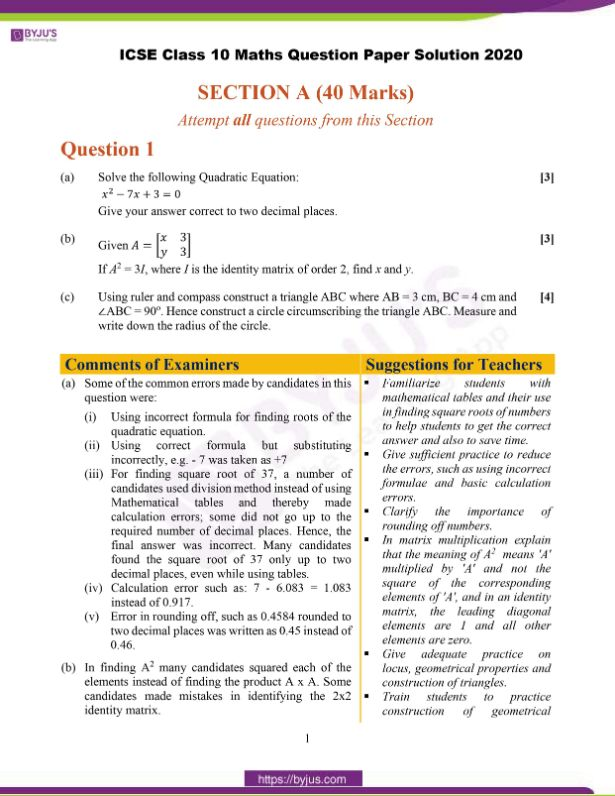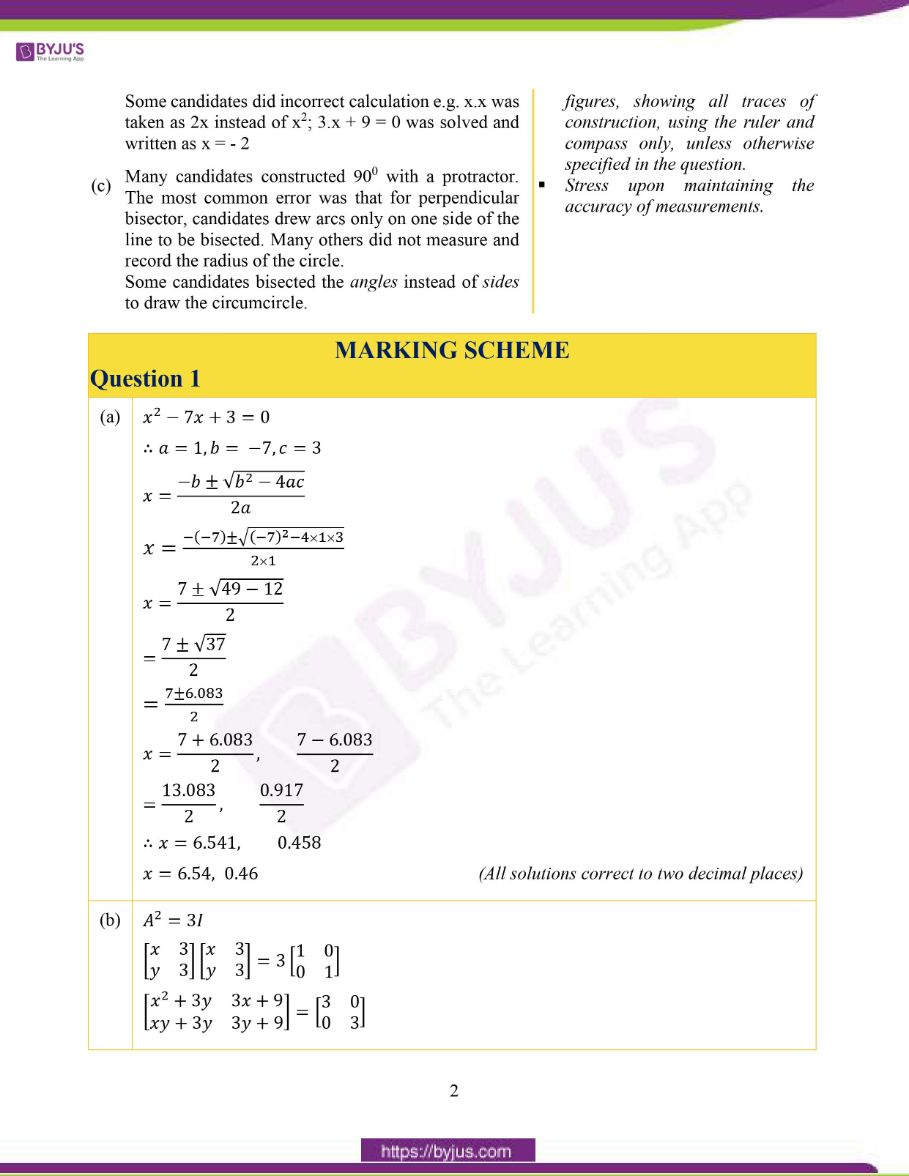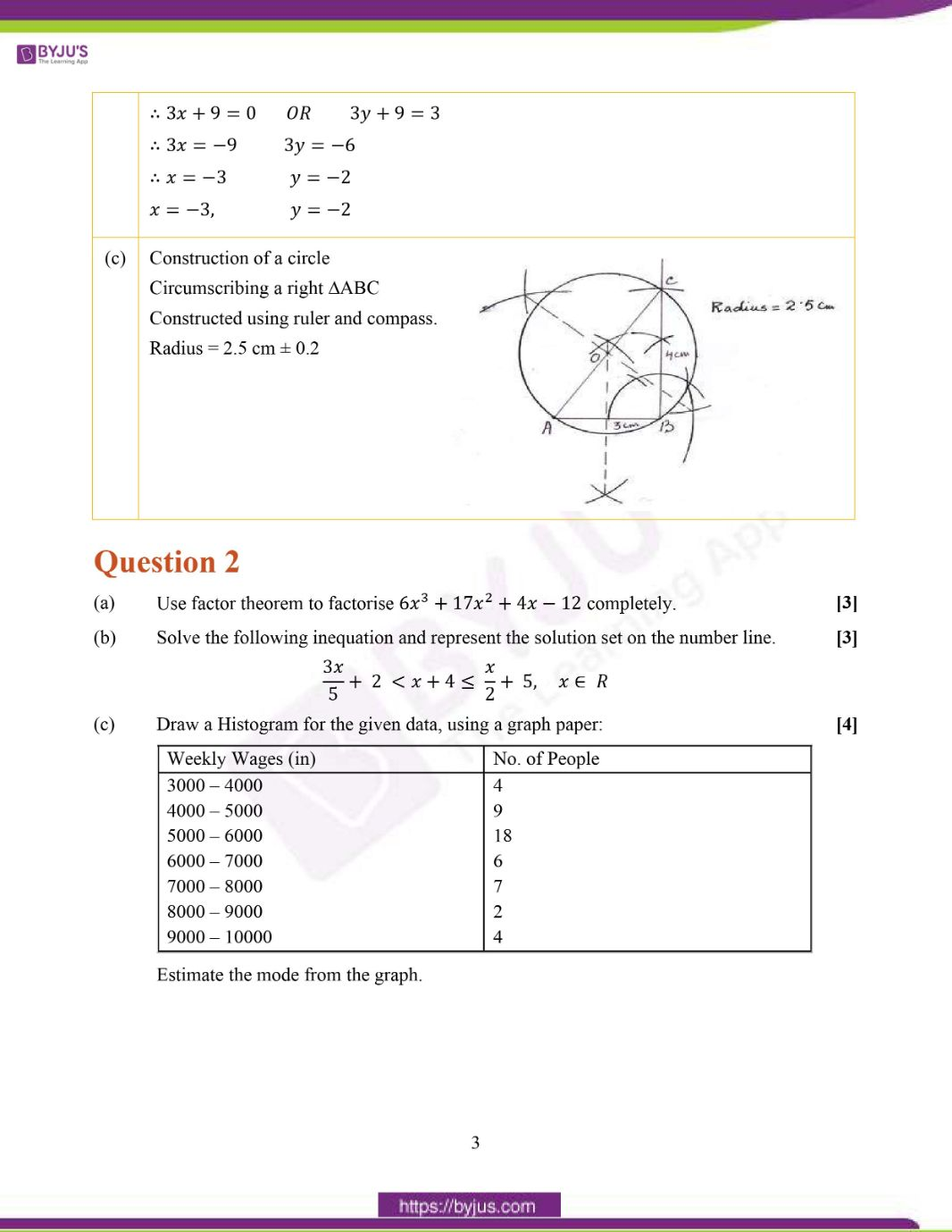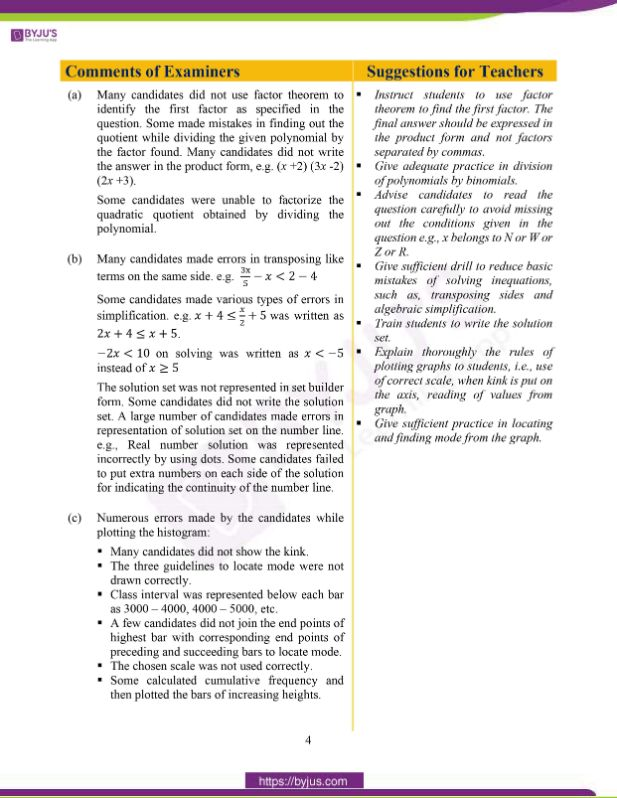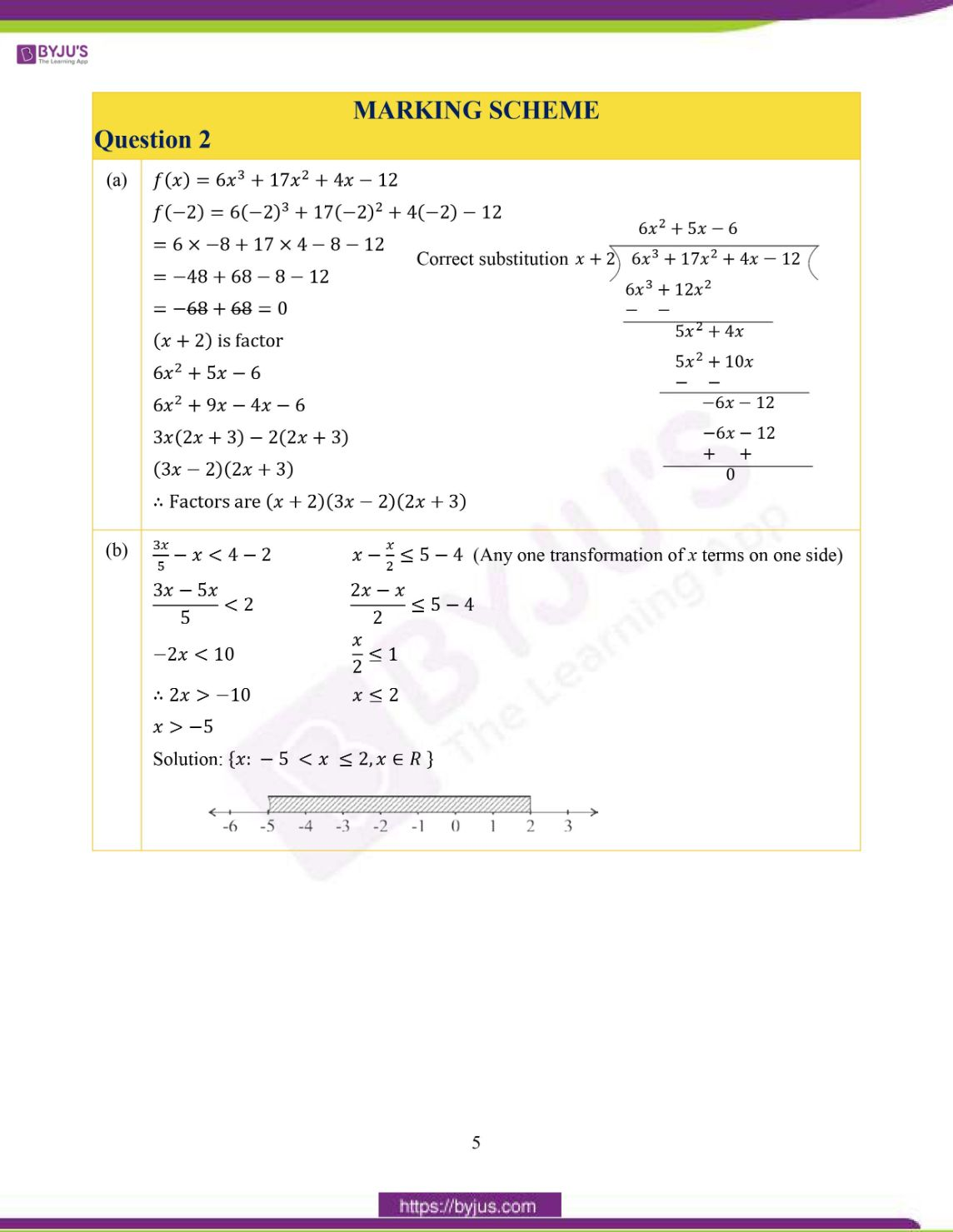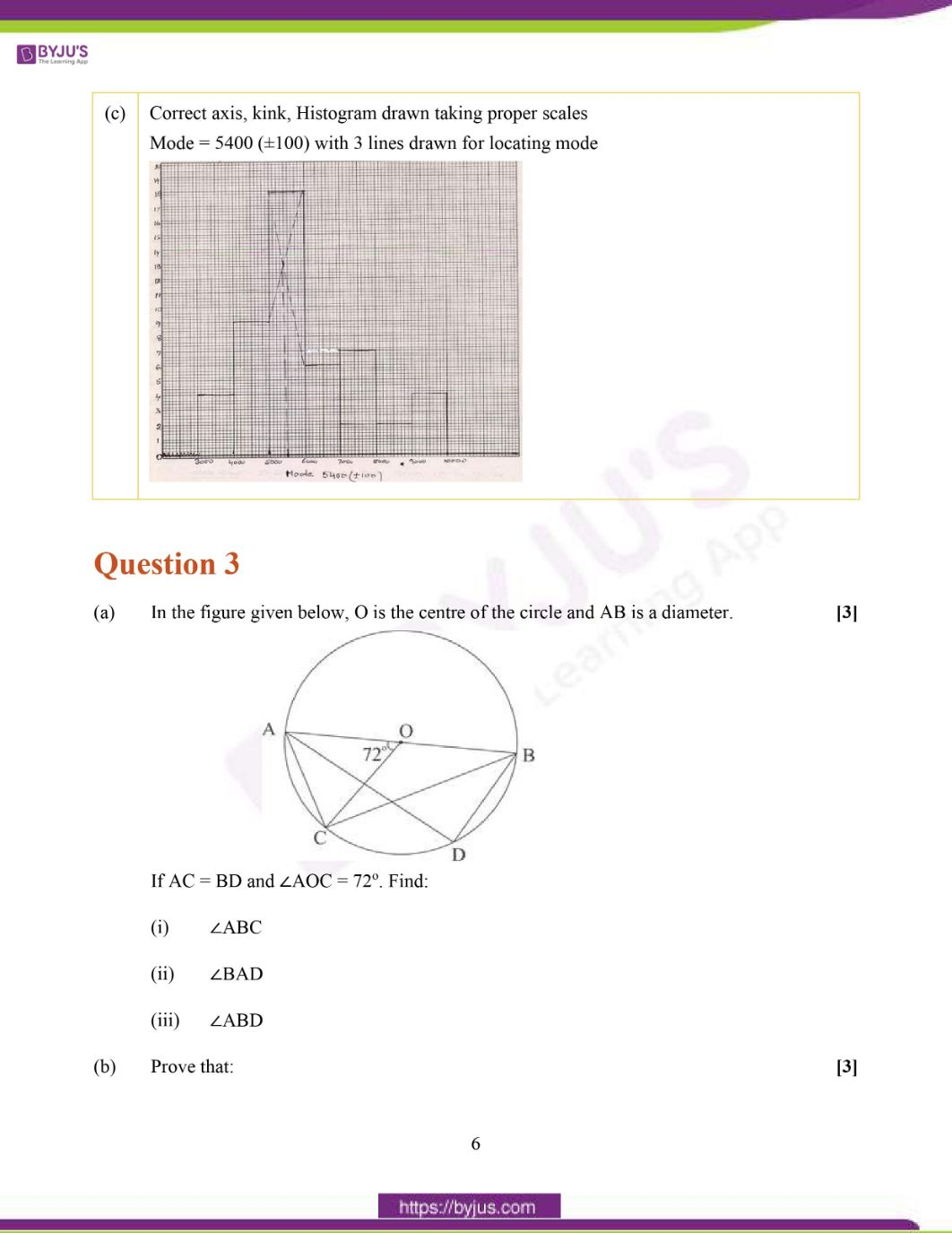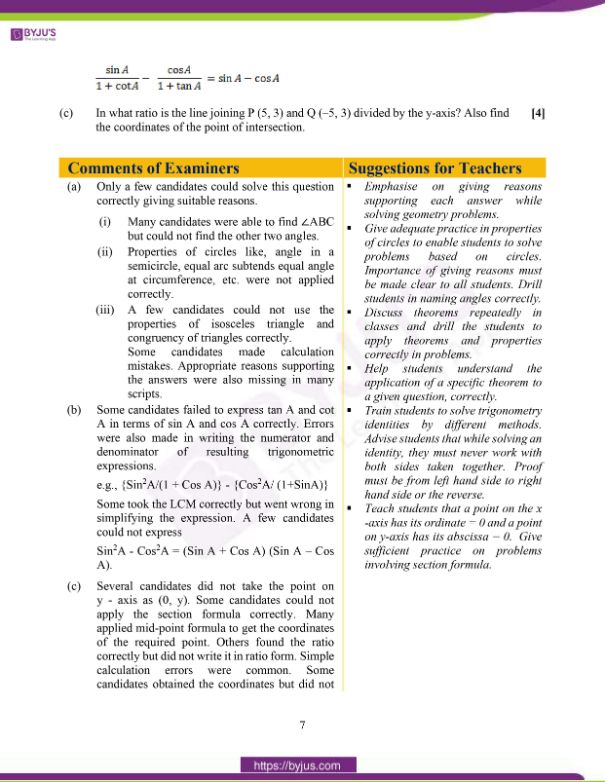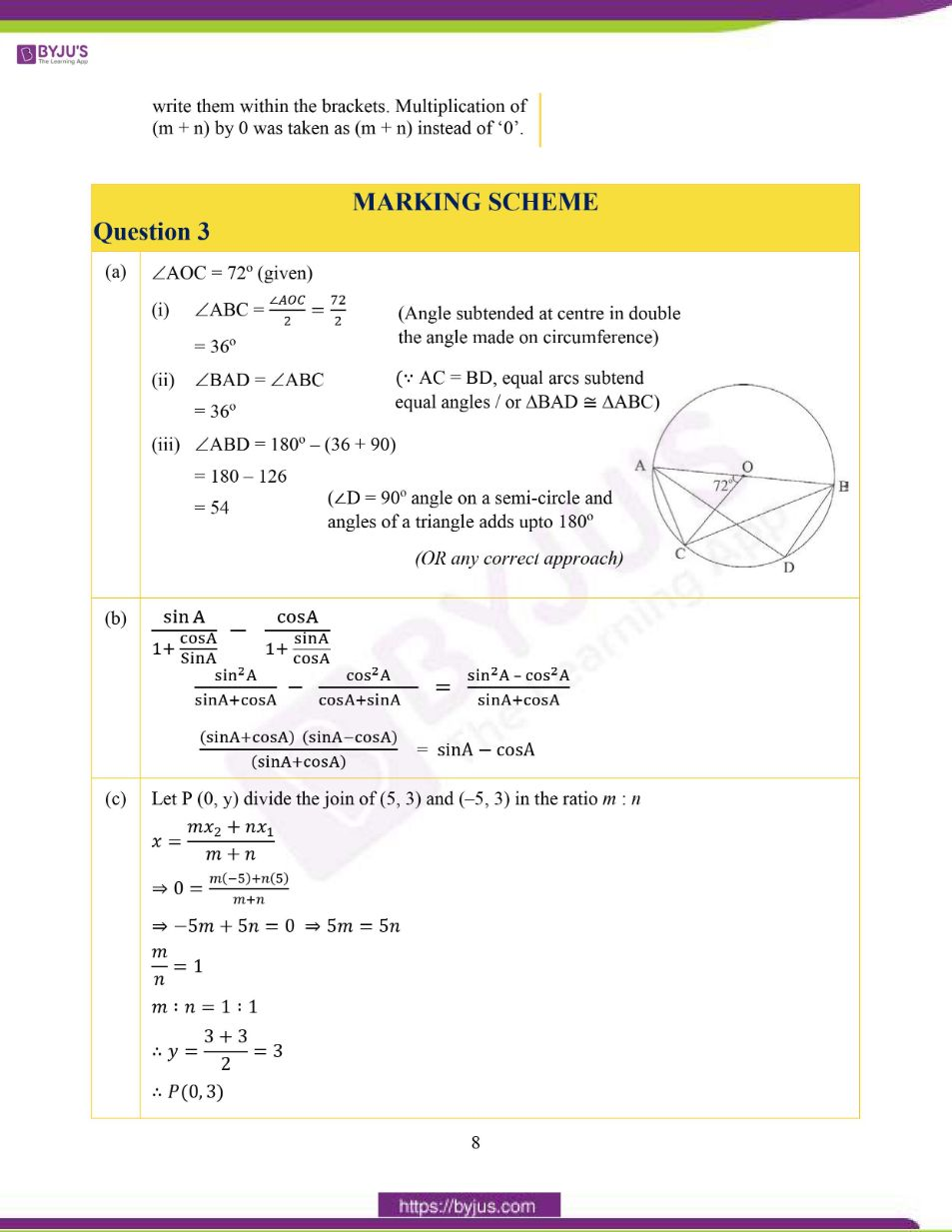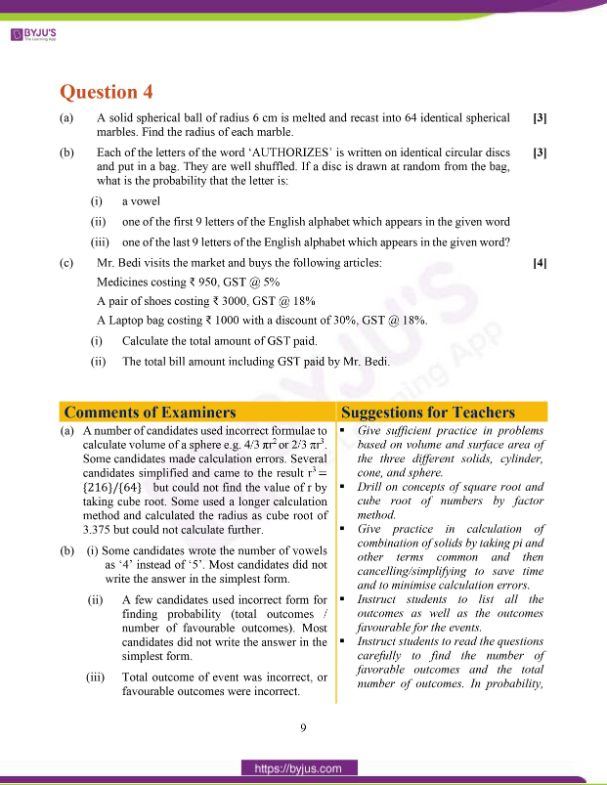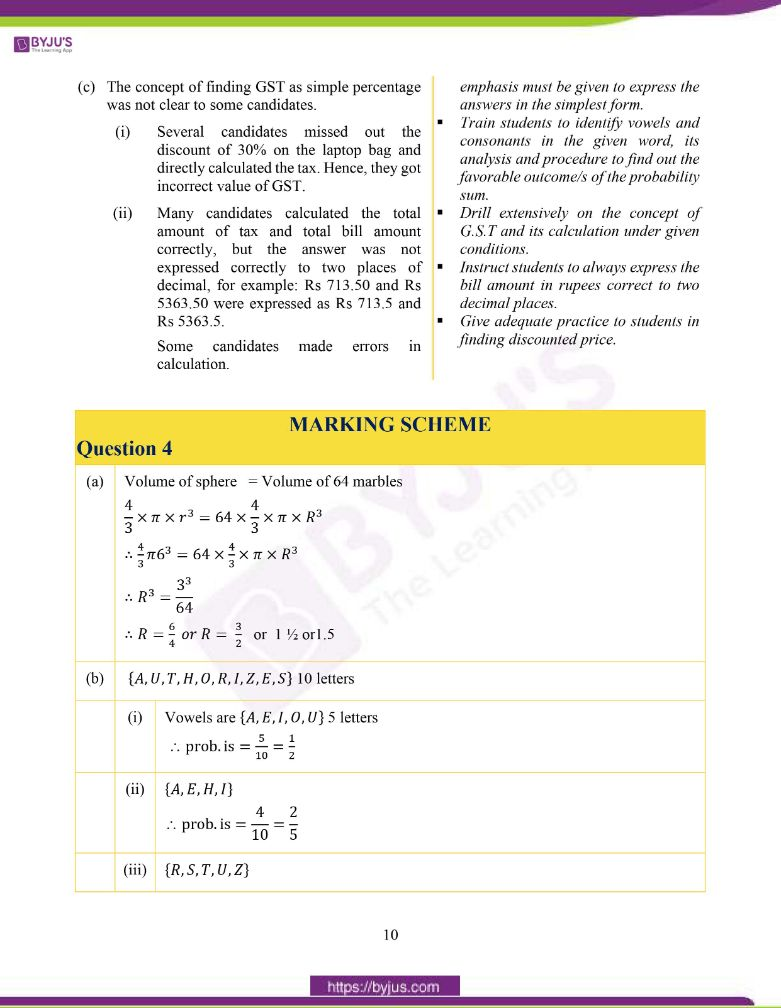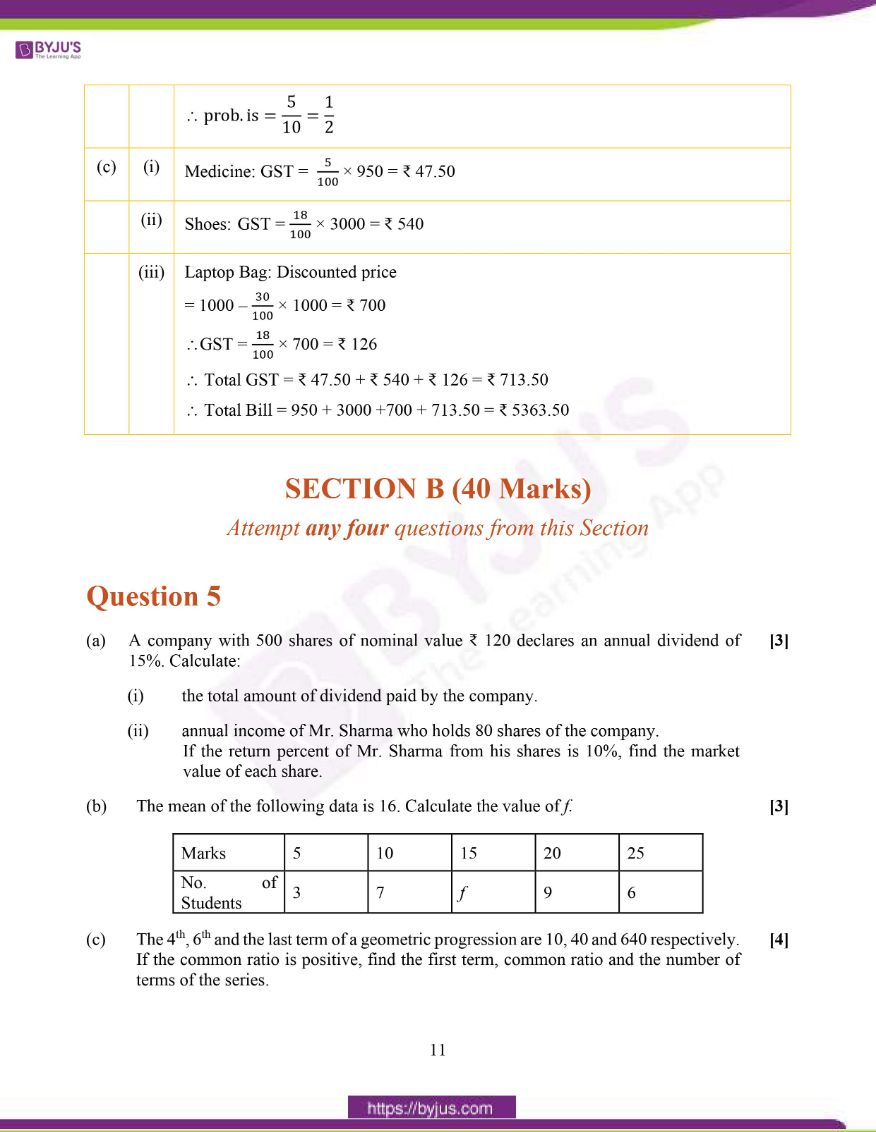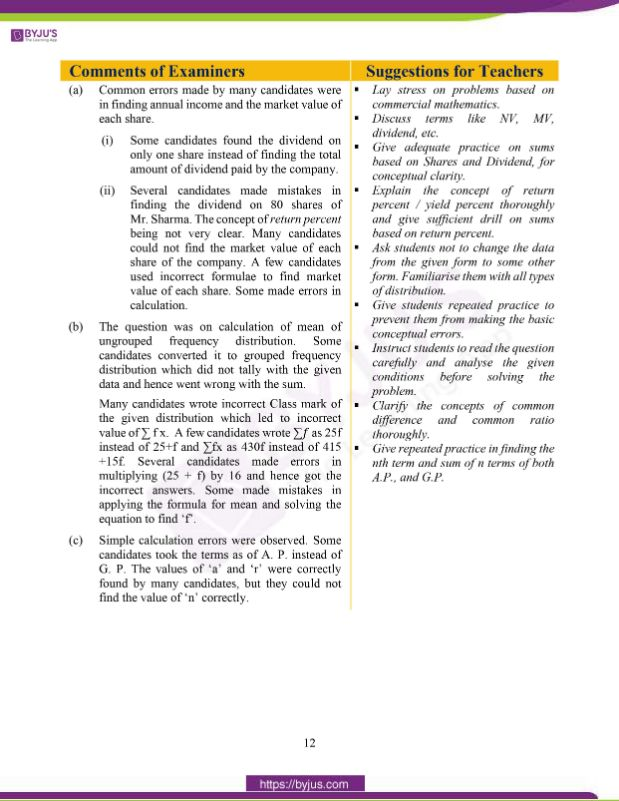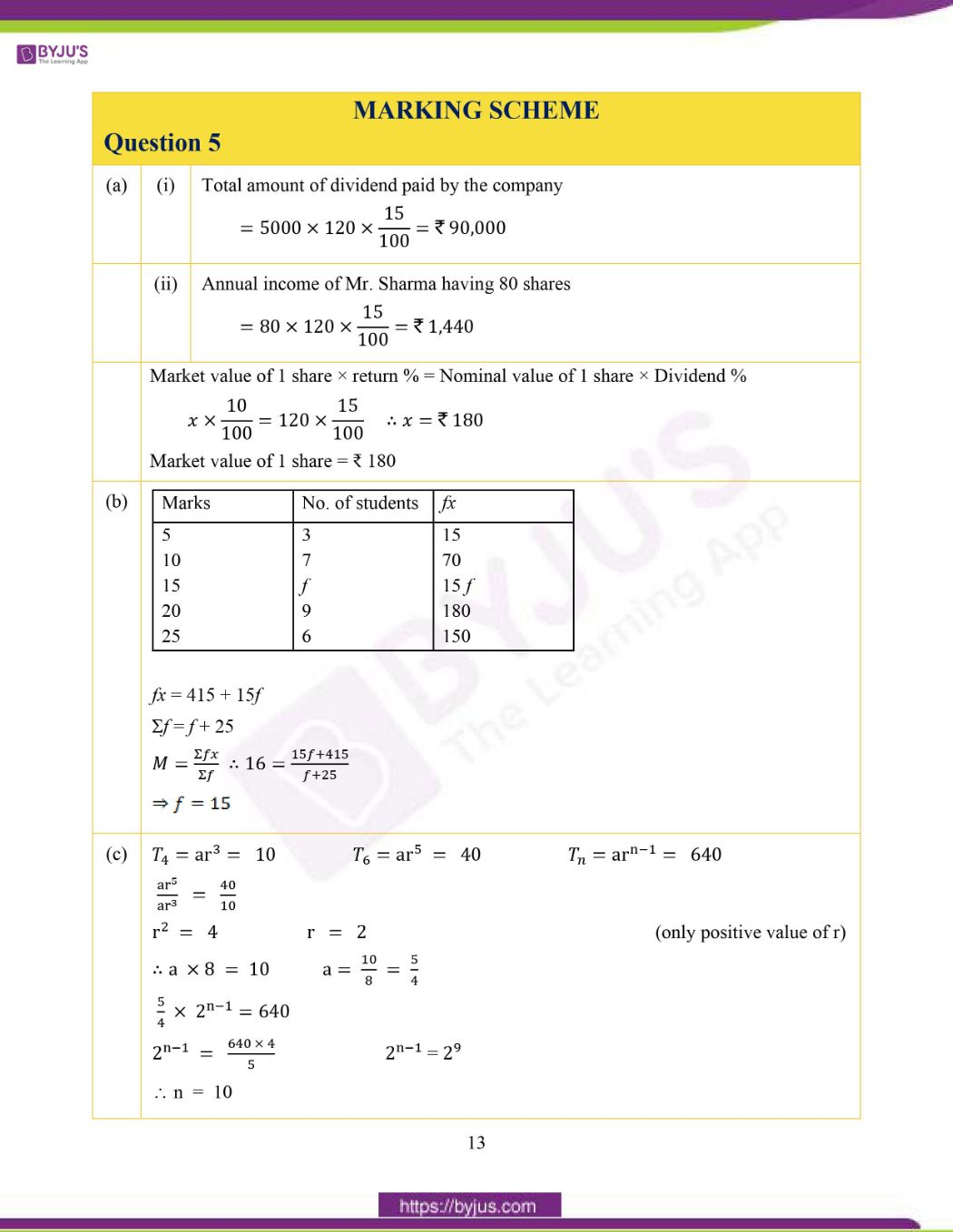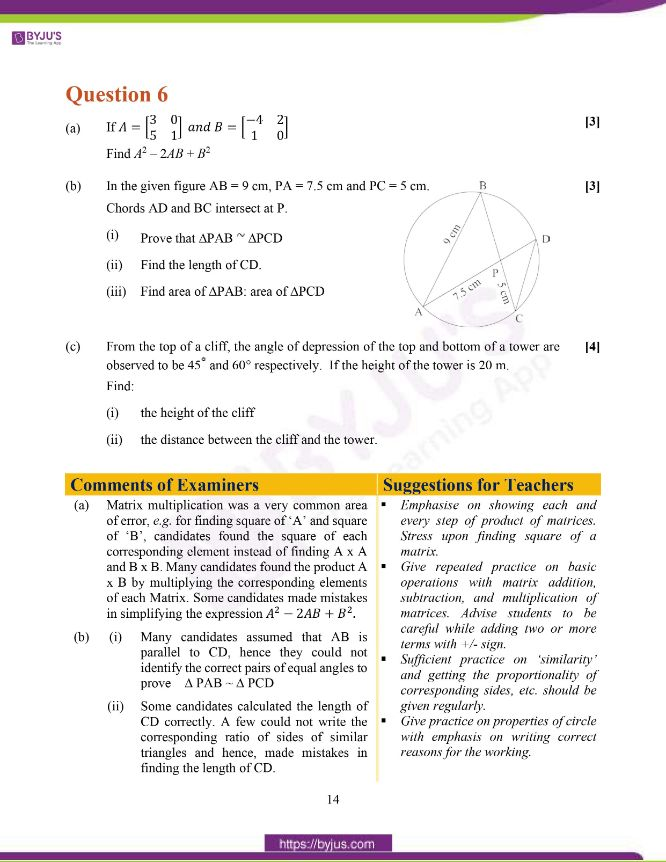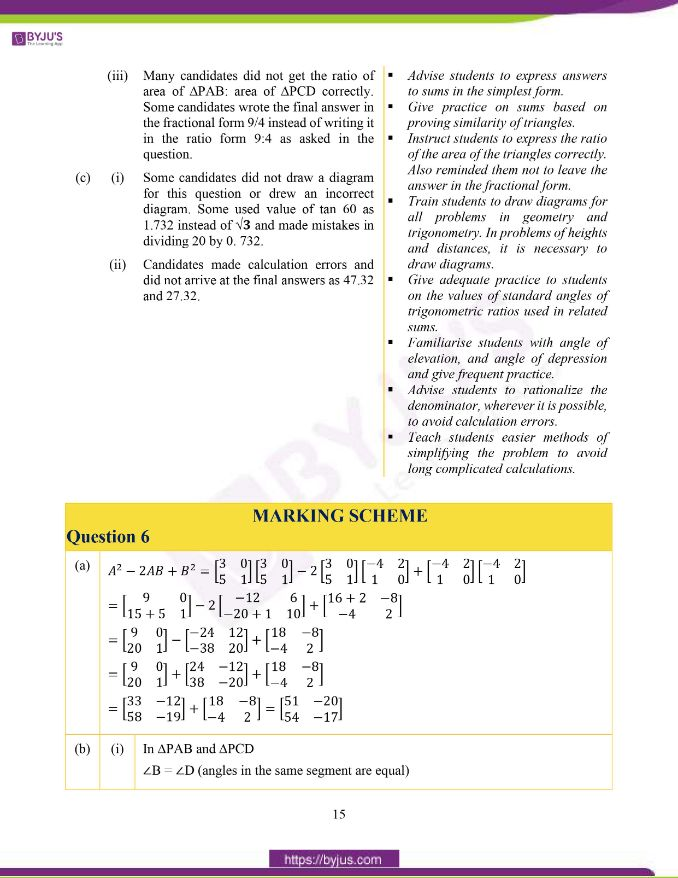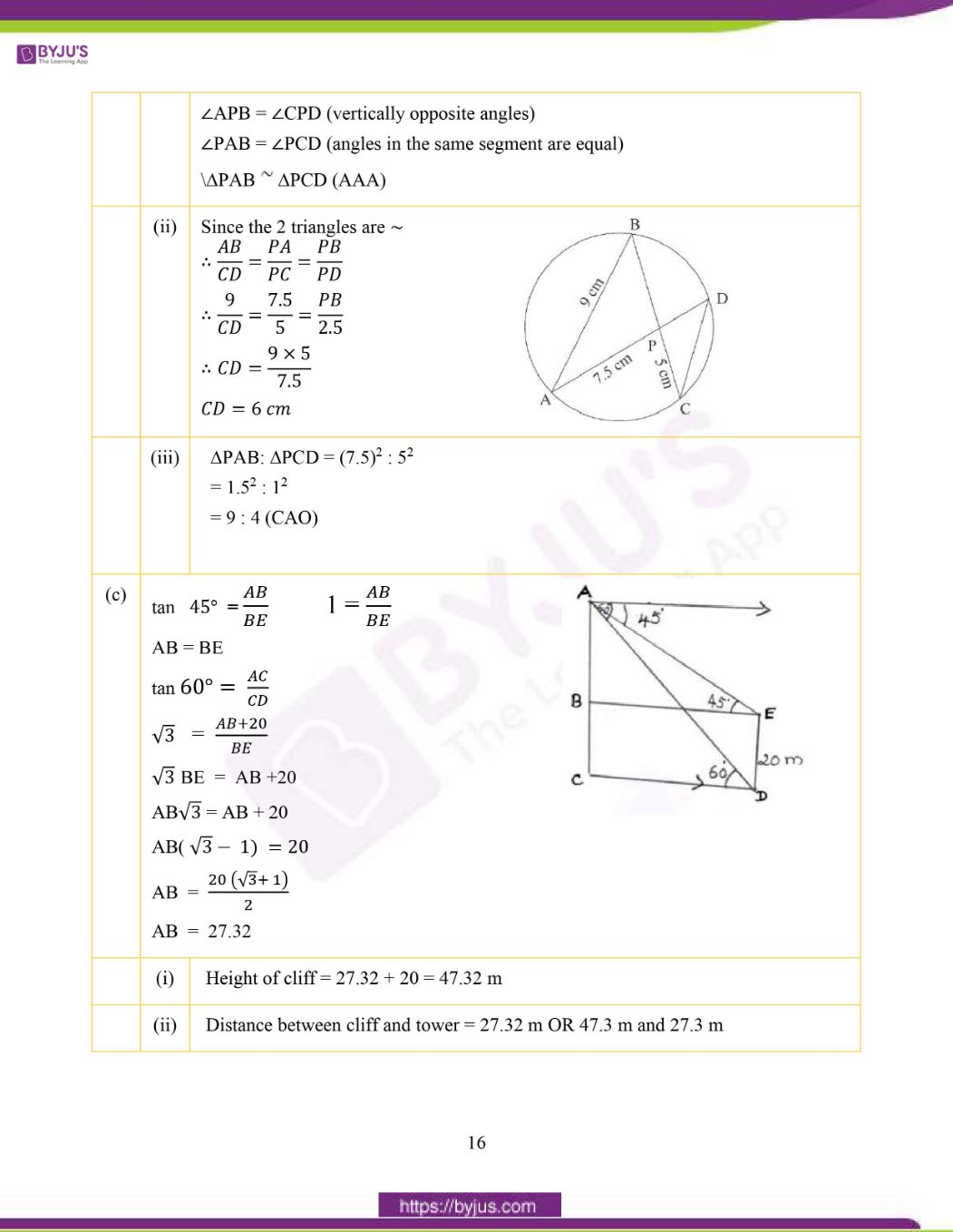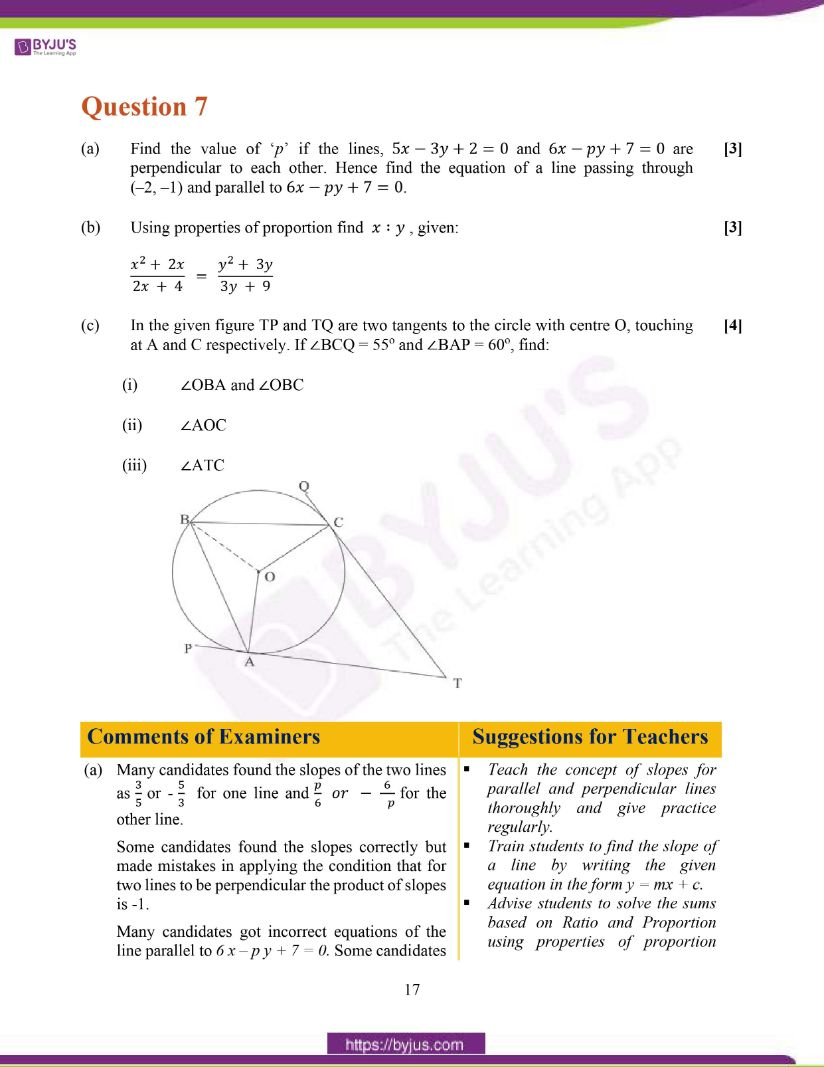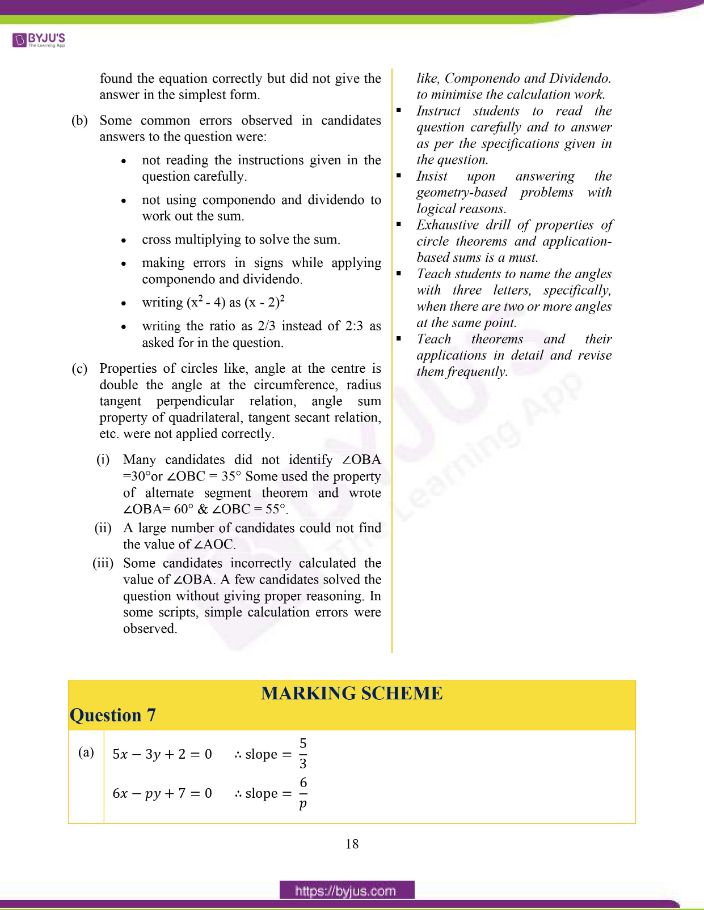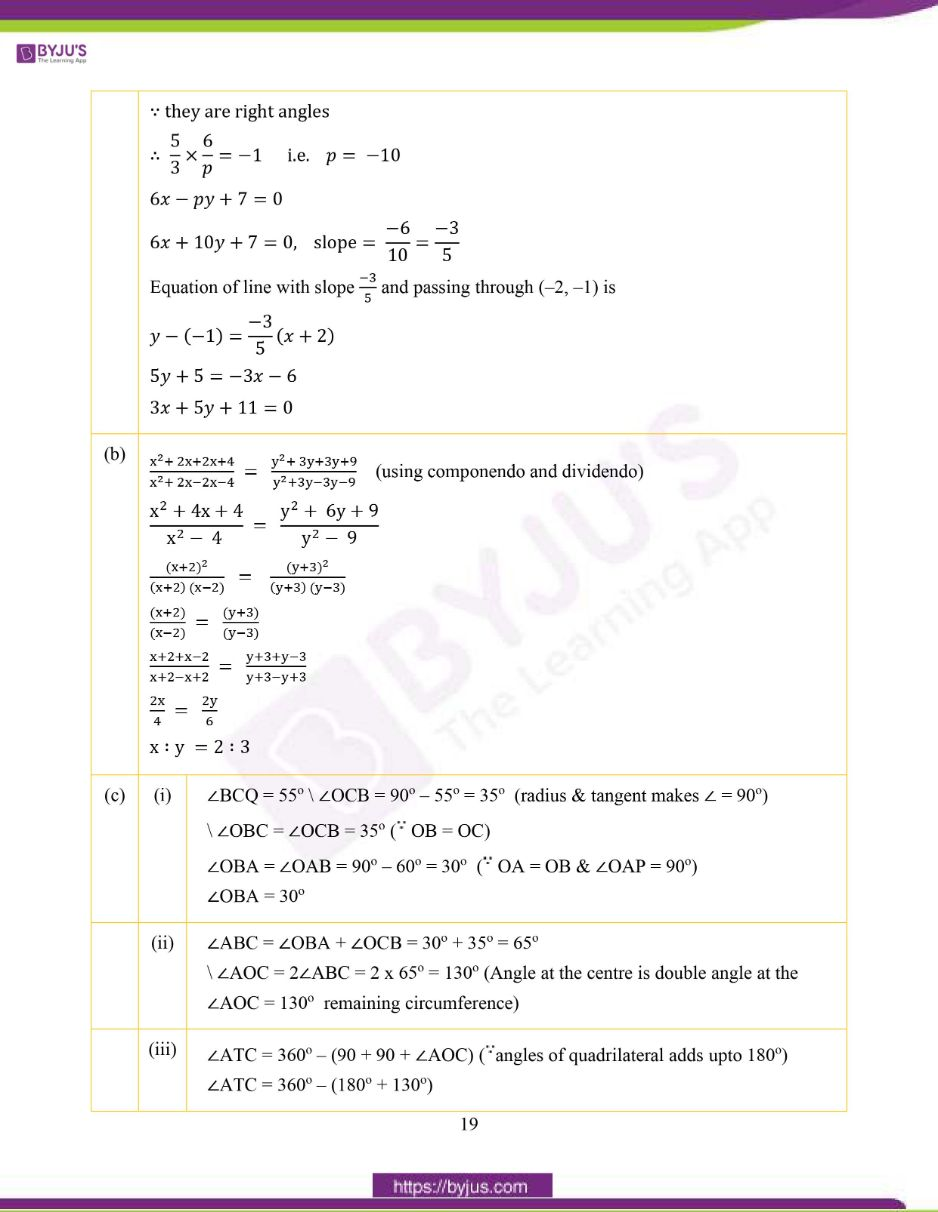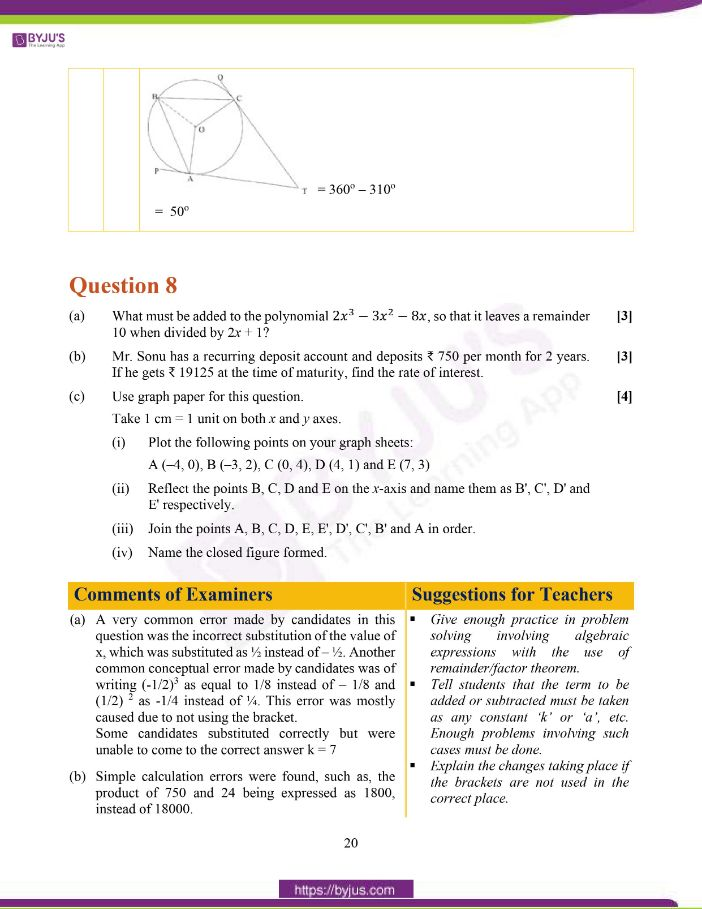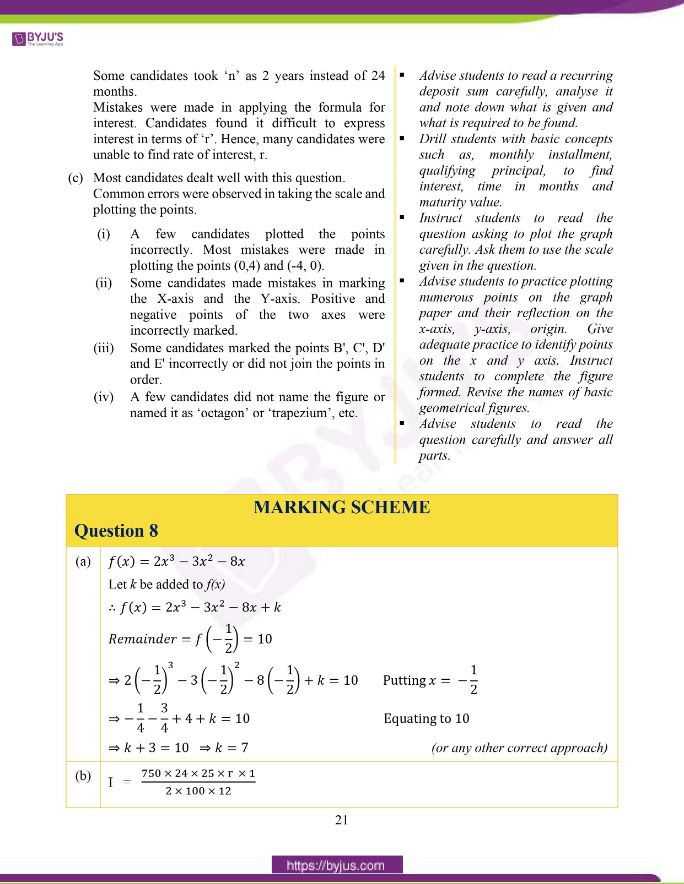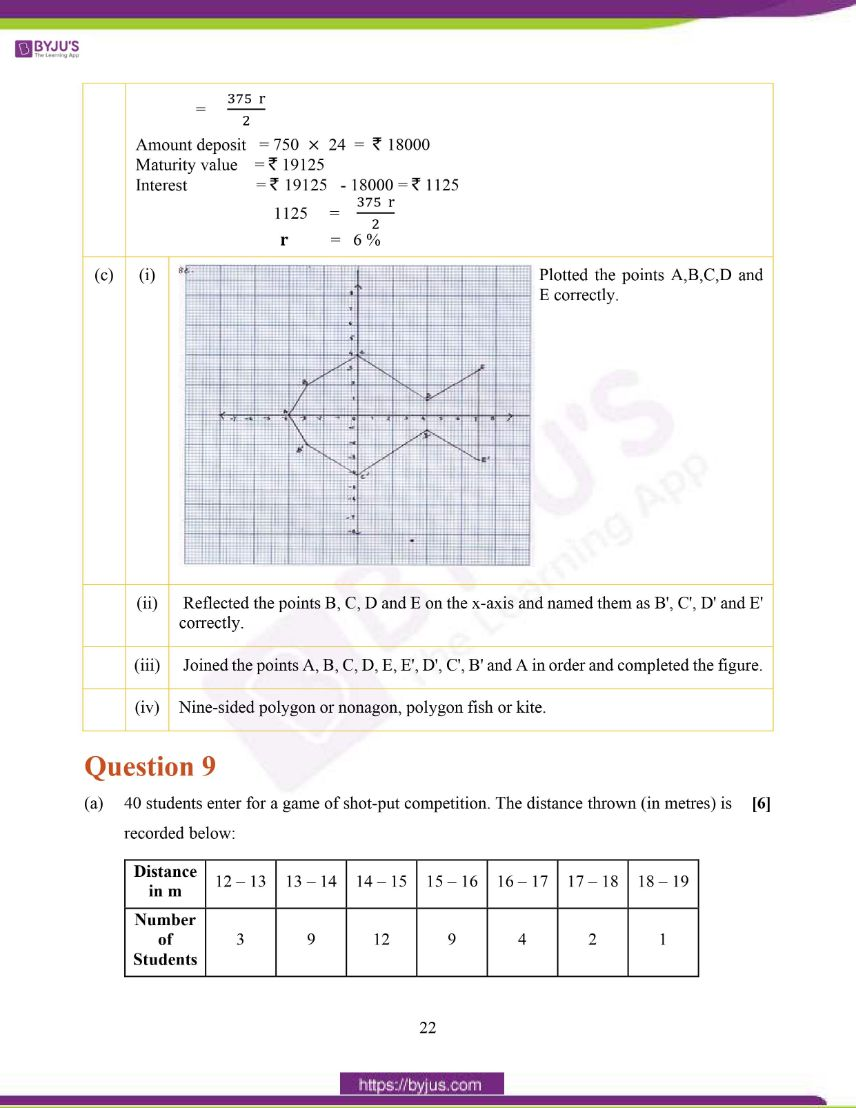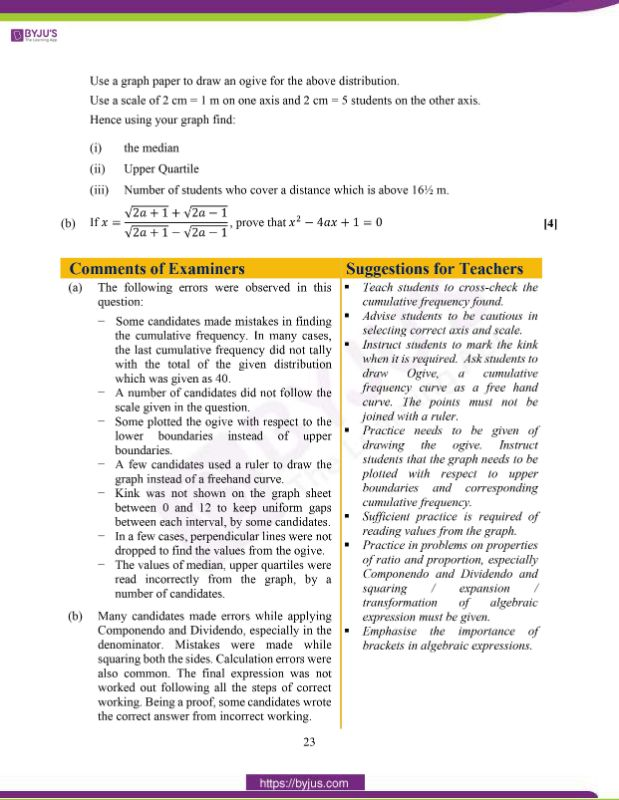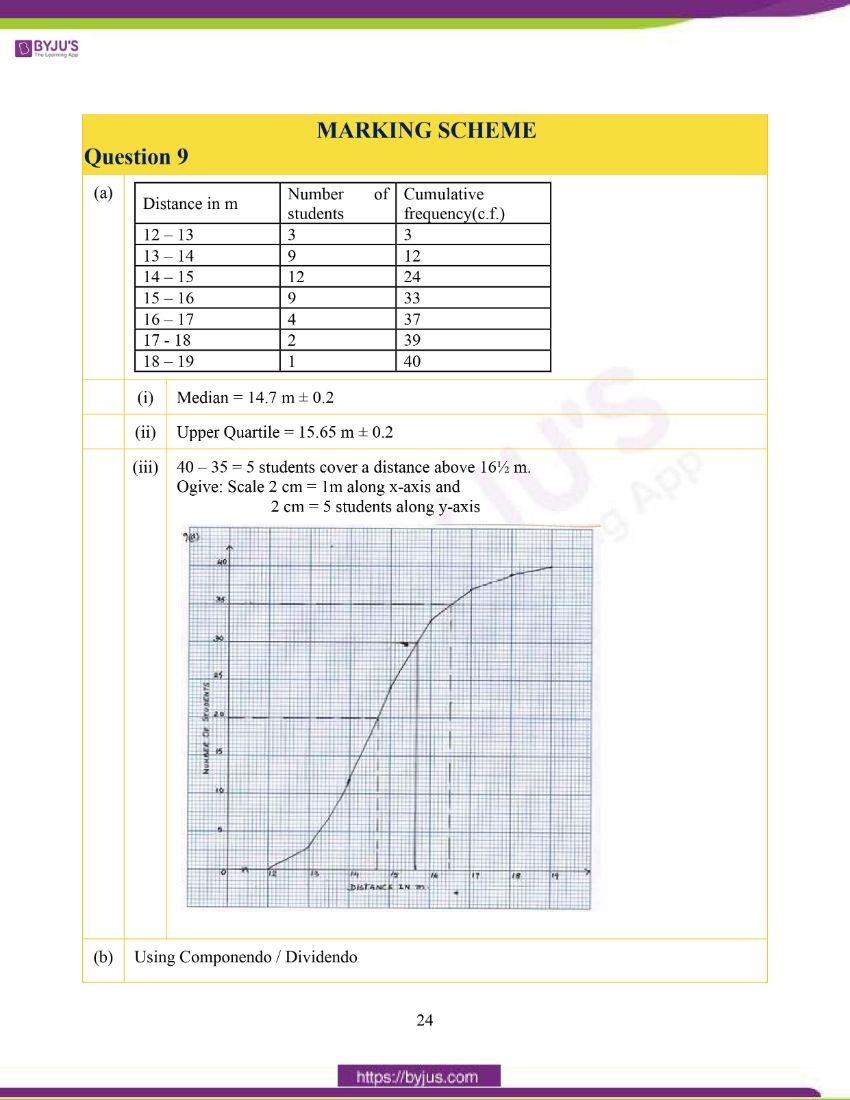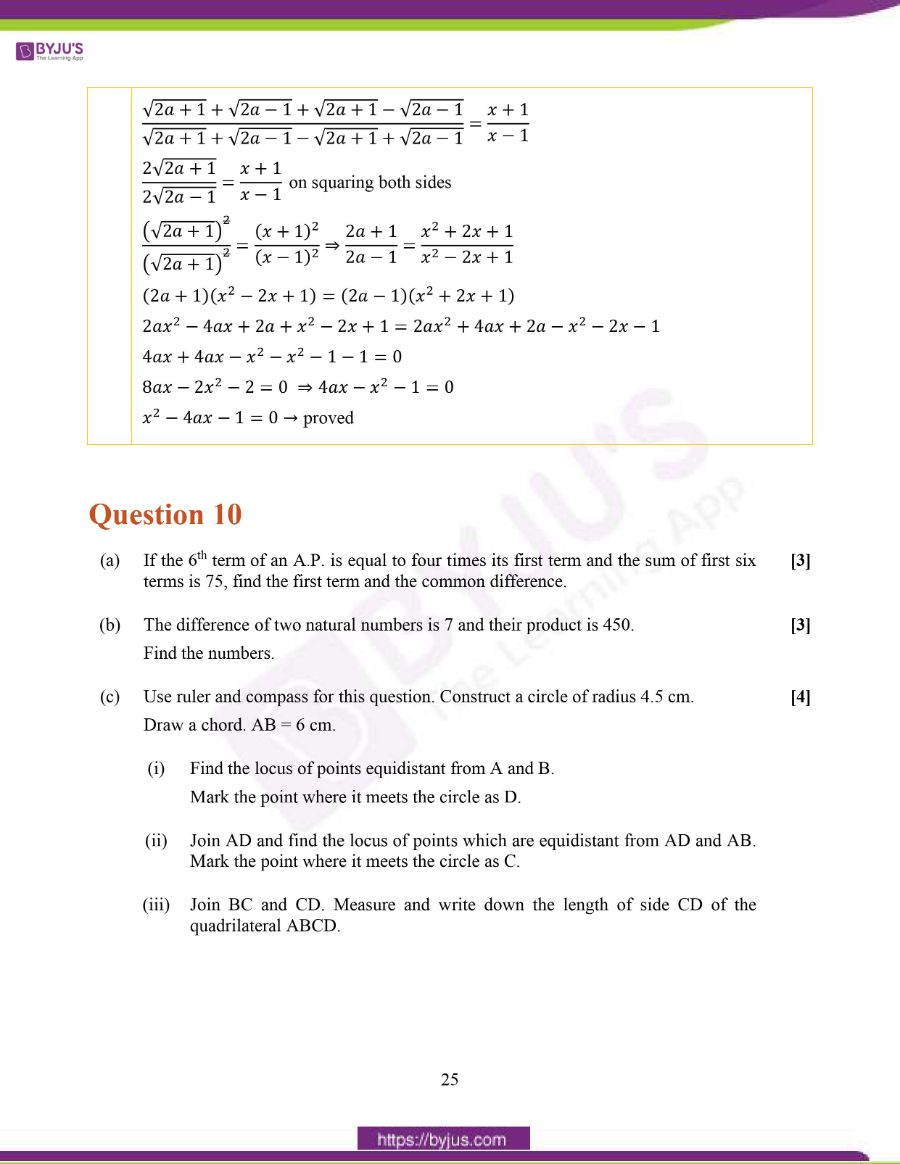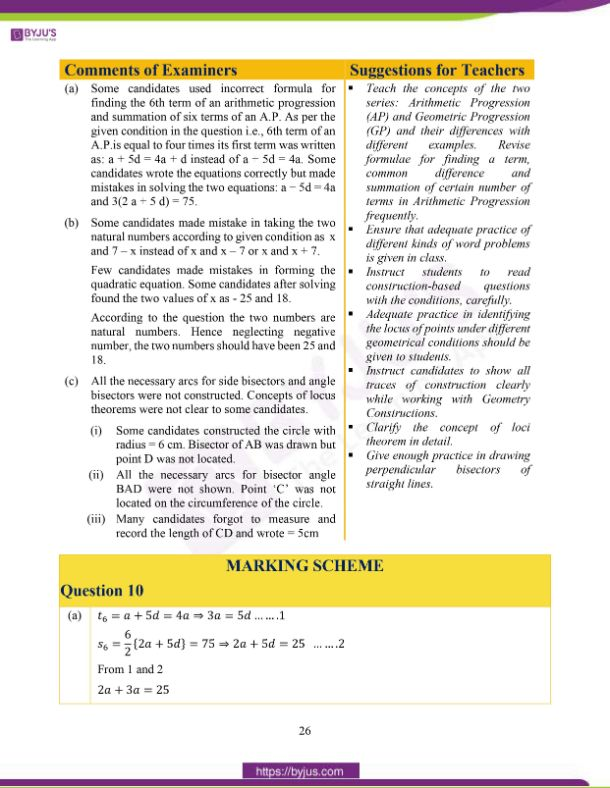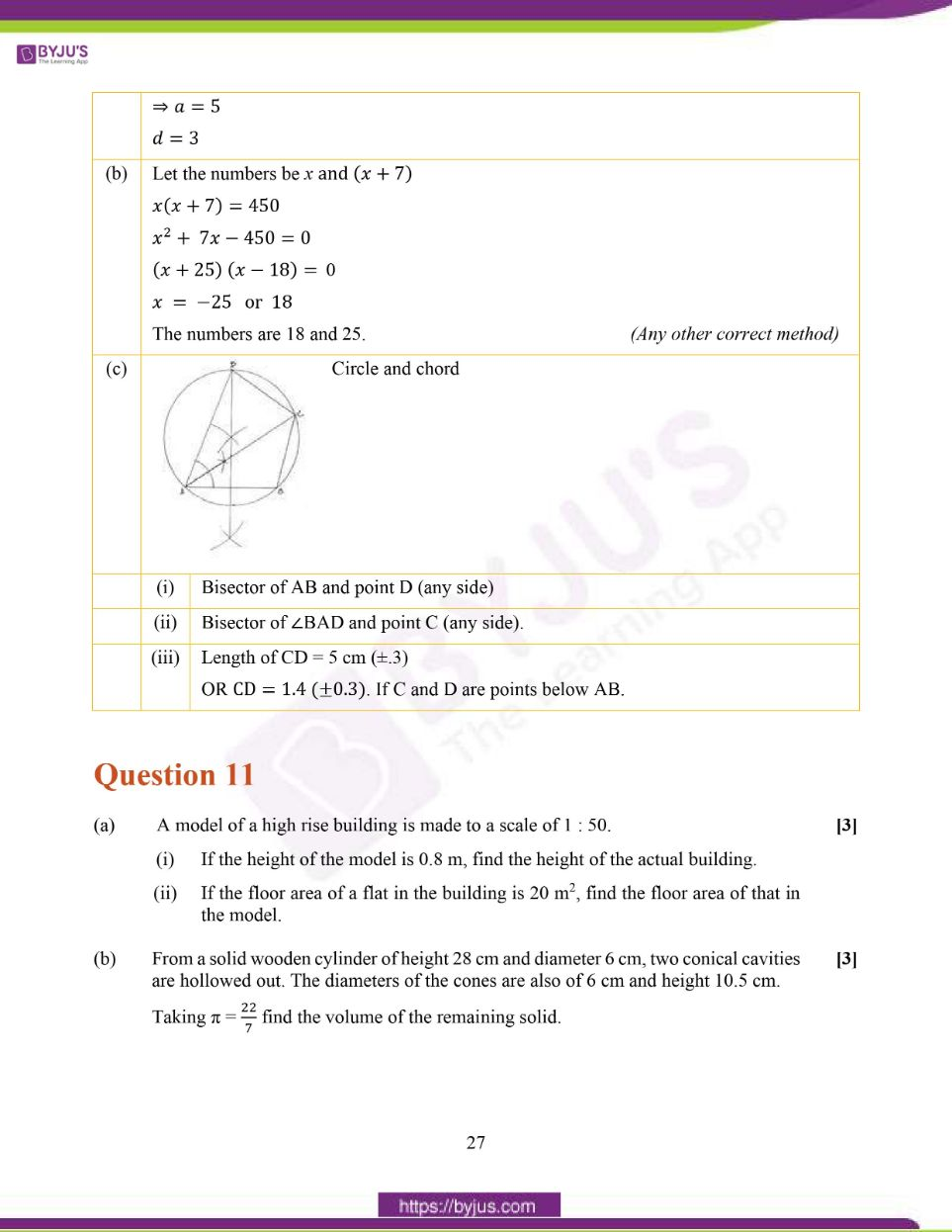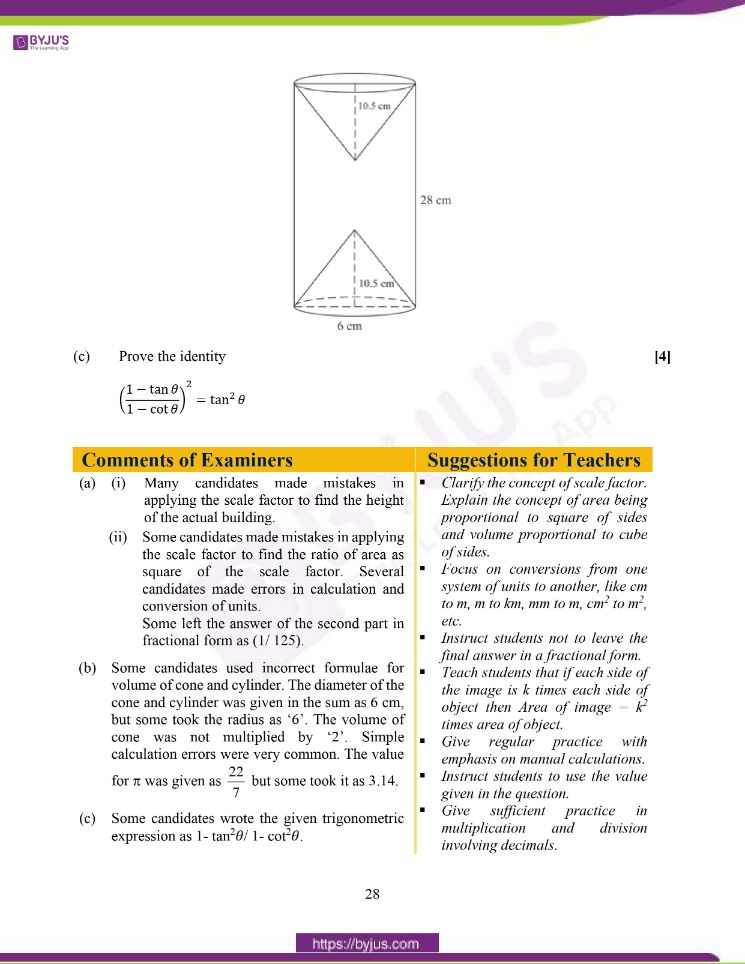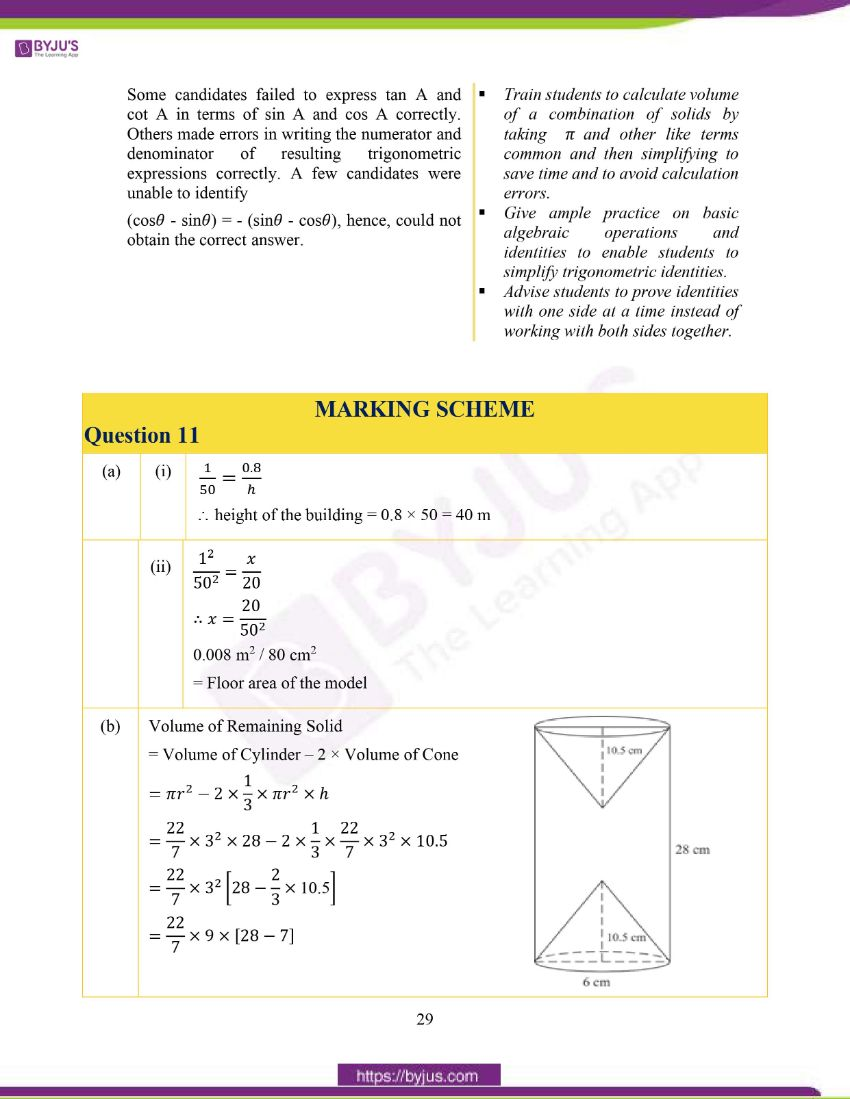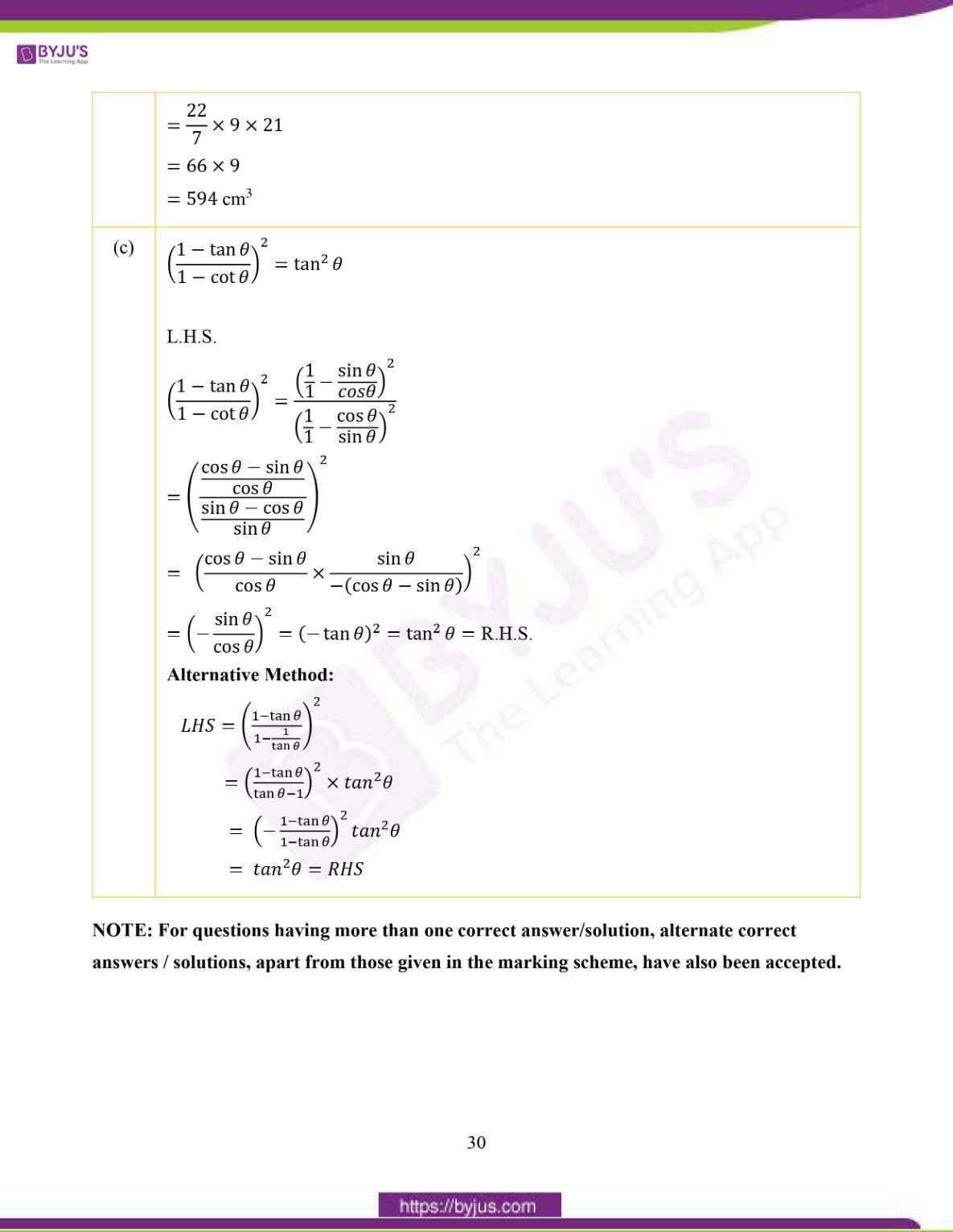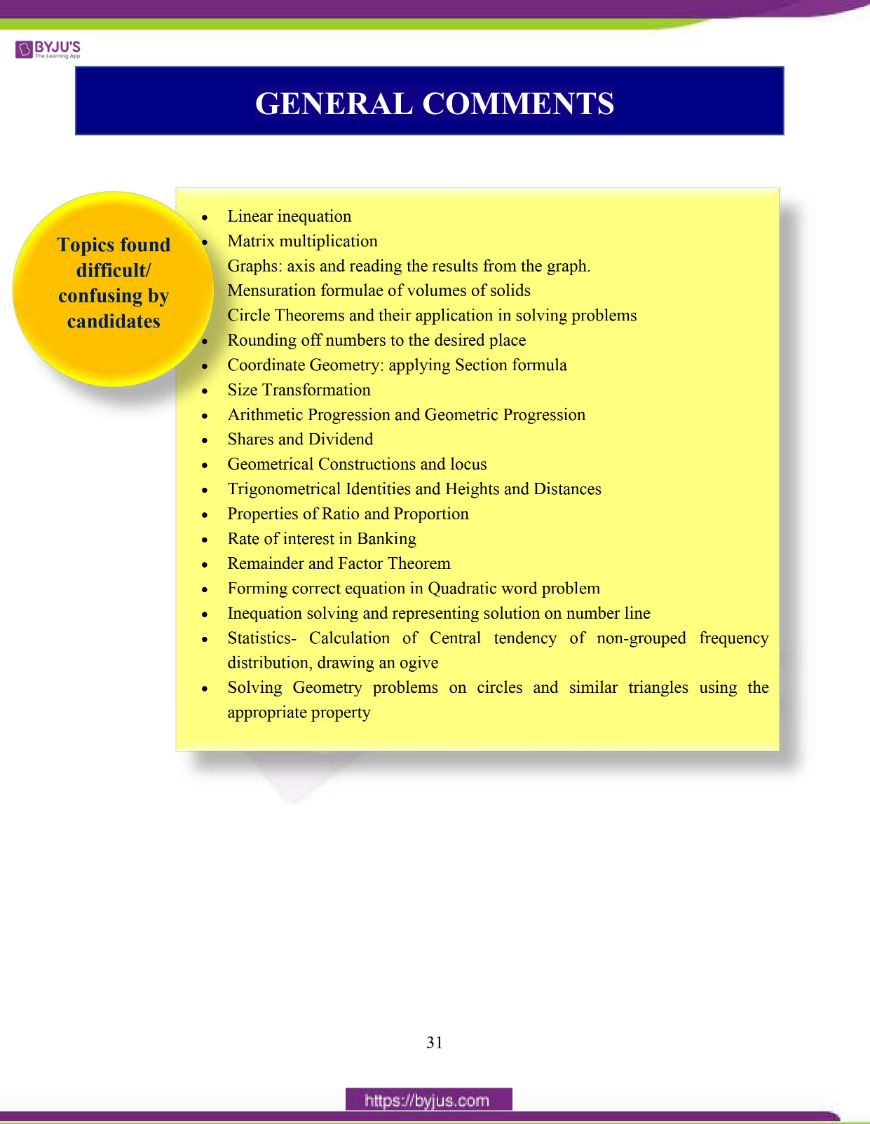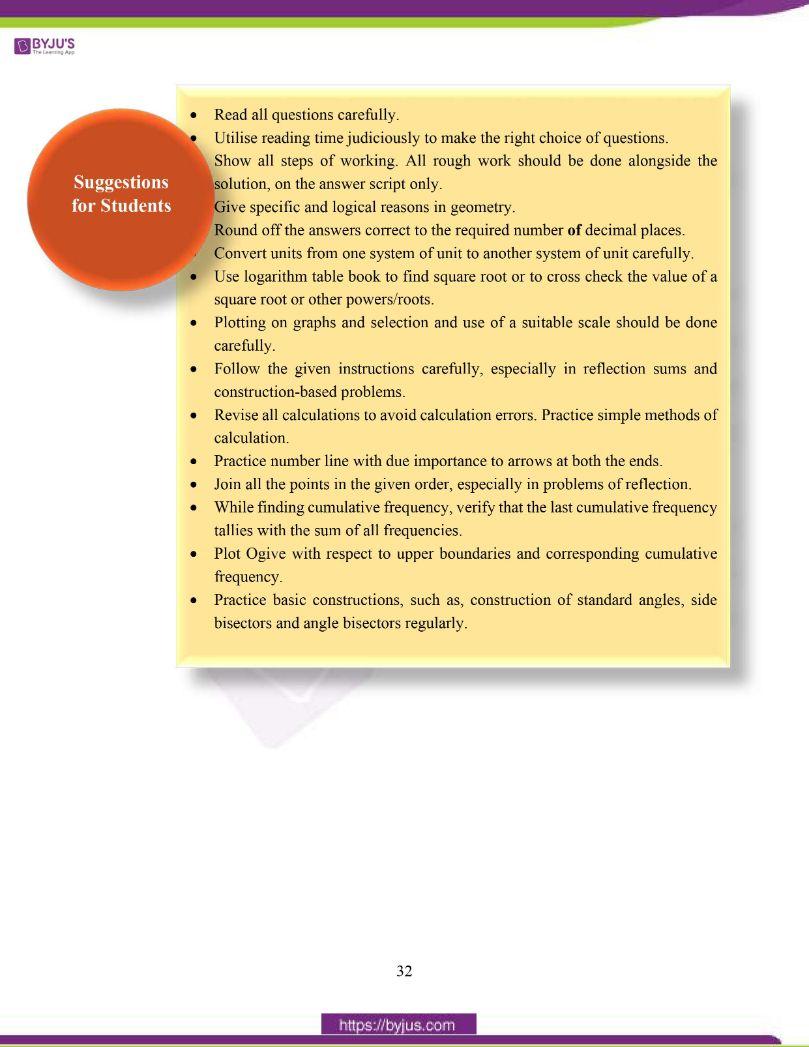### Difficult Topics of ICSE Class 10 Maths 2020 Question Paper

Below we have listed down the topics which students found difficult while attempting the ICSE 2020 Maths Question Paper.

• Linear inequation
• Matrix multiplication
• Graphs: axis and reading the results from the graph.
• Mensuration formulae of volumes of solids
• Circle Theorems and their application in solving problems
• Rounding off numbers to the desired place
• Coordinate Geometry: applying Section formula
• Size Transformation
• Arithmetic Progression and Geometric Progression
• Shares and Dividend
• Geometrical Constructions and locus
• Trigonometric Identities and Heights and Distances
• Properties of Ratio and Proportion
• Rate of interest in Banking
• Remainder and Factor Theorem
• Forming correct equation in Quadratic word problem
• Inequation solving and representing solution on number line
• Statistics- Calculation of Central tendency of non-grouped frequency distribution, drawing an ogive
• Solving Geometry problems on circles and similar triangles using the appropriate property

## ICSE Class 10 Maths Question Paper 2020 With Solutions

### Question 1:

(a) Solve the following Quadratic Equation:

𝑥2 − 7𝑥 + 3 = 0

(b) GivenIf A2 = 3I, where I is the identity matrix of order 2, find x and y.

(c) Using ruler and compass construct a triangle ABC where AB = 3 cm, BC = 4 cm and ∠ABC = 90o. Hence construct a circle circumscribing the triangle ABC. Measure and write down the radius of the circle.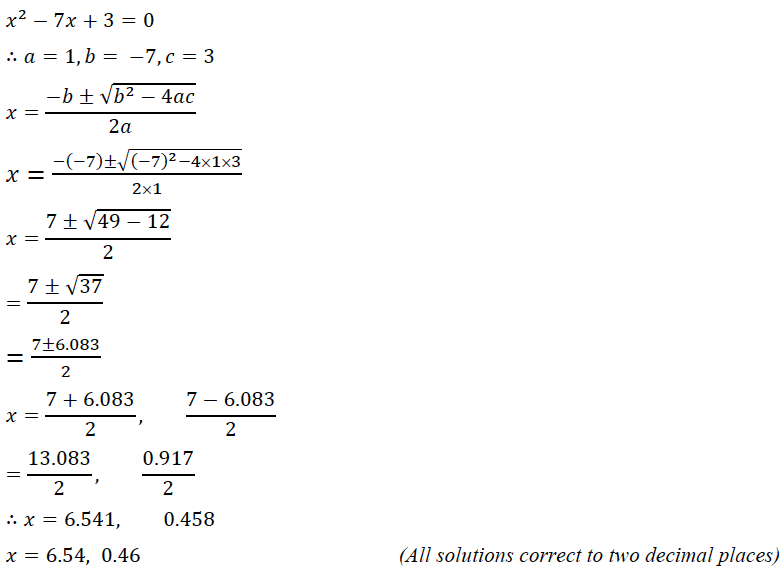(b)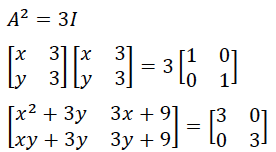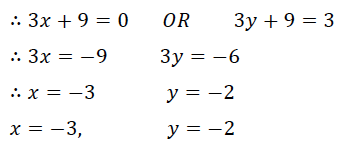(c)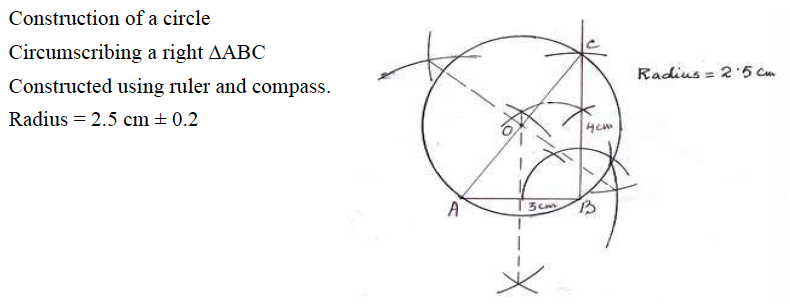### Question 2:

(a) Use factor theorem to factorise 6𝑥3 + 17𝑥2 + 4𝑥 − 12 completely.

(b) Solve the following inequation and represent the solution set on the number line.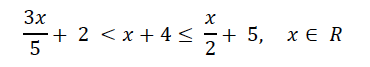(c) Draw a Histogram for the given data, using a graph paper:

 Weekly Wages (in) No. of People 3000 – 4000 4 4000 – 5000 9 5000 – 6000 18 6000 – 7000 6 7000 – 8000 7 8000 – 9000 2 9000 – 10000 4

Estimate the mode from the graph.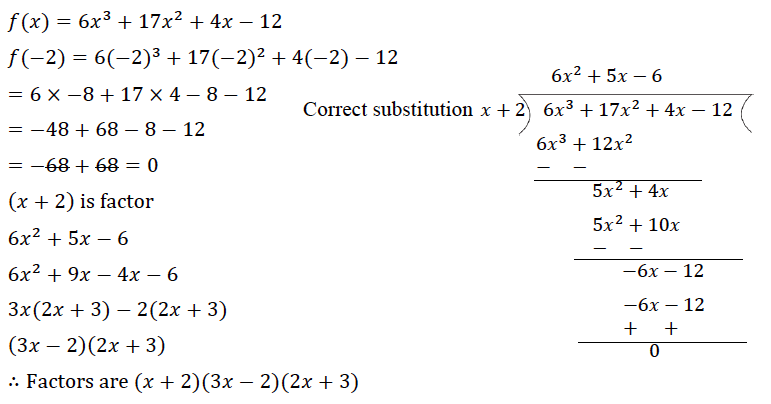(b)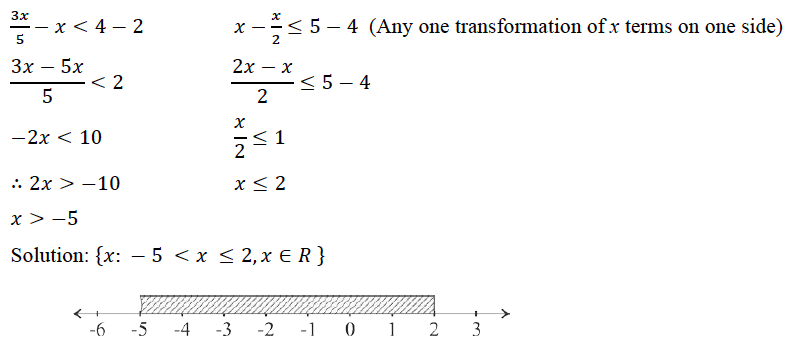(c)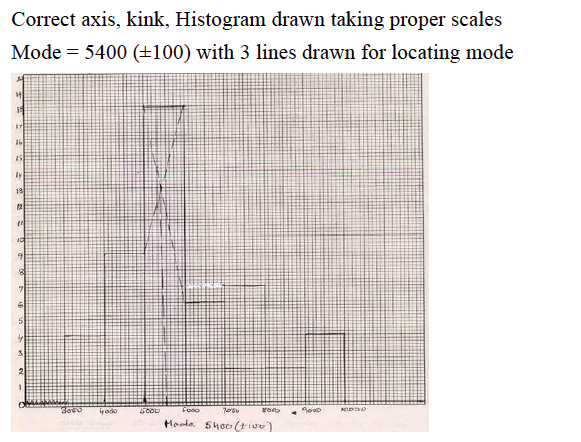### Question 3:

(a) In the figure given below, O is the centre of the circle and AB is a diameter.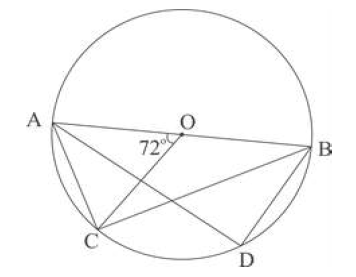If AC = BD and ∠AOC = 72o. Find:

(i) ∠ABC

(iii) ∠ABD

(b) Prove that: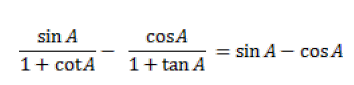(c) In what ratio is the line joining P (5, 3) and Q (–5, 3) divided by the y-axis? Also find the coordinates of the point of intersection.

Answer: (a) ∠AOC = 72o (given)(i) ∠ABC = ∠AOC/2 = 72/2 (Angle subtended at centre in double the angle made on circumference)

= 36o

(∵ AC = BD, equal arcs subtend equal angles / or ΔBAD ≅ ΔABC)

= 36o

(iii) ∠ABD = 180o – (36 + 90)

(∠D = 90o angle on a semi-circle and angles of a triangle adds up to 180o

= 180 – 126

= 54

(b)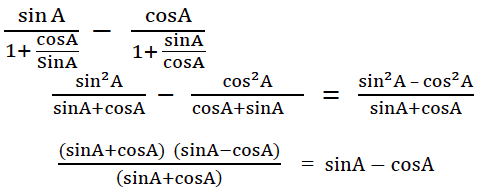(c)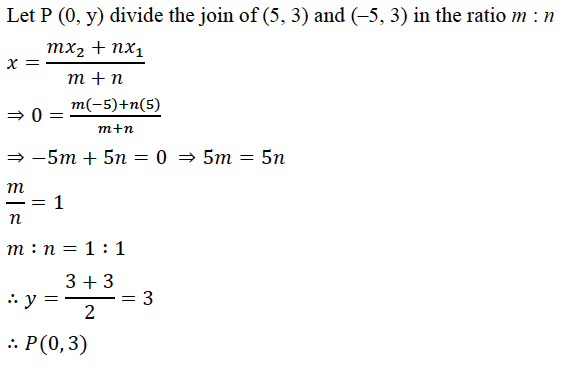### Question 4:

(a) A solid spherical ball of radius 6 cm is melted and recast into 64 identical spherical marbles. Find the radius of each marble.

(b) Each of the letters of the word ‘AUTHORIZES’ is written on identical circular discs and put in a bag. They are well shuffled. If a disc is drawn at random from the bag, what is the probability that the letter is:

(i) a vowel

(ii) one of the first 9 letters of the English alphabet which appears in the given word

(iii) one of the last 9 letters of the English alphabet which appears in the given word?

(c) Mr. Bedi visits the market and buys the following articles:

Medicines costing ₹ 950, GST @ 5%

A pair of shoes costing ₹ 3000, GST @ 18%

A Laptop bag costing ₹ 1000 with a discount of 30%, GST @ 18%.

(i) Calculate the total amount of GST paid.

(ii) The total bill amount including GST paid by Mr. Bedi.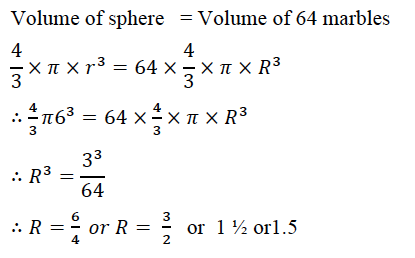(b) {𝐴, 𝑈, 𝑇, 𝐻, 𝑂, 𝑅, 𝐼, 𝑍, 𝐸, 𝑆} 10 letters

(i) Vowels are {𝐴, 𝐸, 𝐼, 𝑂, 𝑈} 5 letters

∴ prob. is = 5/10 = 1/2

(ii) {𝐴, 𝐸, 𝐻, 𝐼}

∴ prob. is = 4/10 = 2/5

(iii) {𝑅, 𝑆, 𝑇, 𝑈, 𝑍}

∴ prob. is = 5/10 = 1/2

(c) (i) Medicine: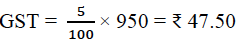(ii) Shoes: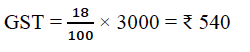(iii) Laptop Bag: Discounted price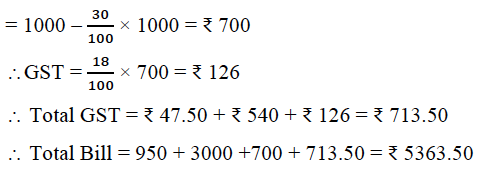### Question 5:

(a) A company with 500 shares of nominal value ₹ 120 declares an annual dividend of 15%. Calculate:

(i) the total amount of dividend paid by the company.

(ii) annual income of Mr. Sharma who holds 80 shares of the company. If the return percent of Mr. Sharma from his shares is 10%, find the market value of each share.

(b) The mean of the following data is 16. Calculate the value of f.

 Marks 5 10 15 20 25 No. of Students 3 7 f 9 6

(c) The 4th, 6th and the last term of a geometric progression are 10, 40 and 640, respectively. If the common ratio is positive, find the first term, common ratio and the number of terms of the series.

(a) (i) Total amount of dividend paid by the company

= 5000 × 120 × 15/100 = Rs. 90,000

(ii) Annual income of Mr. Sharma having 80 shares

= 80 × 120 × 15/100 = Rs. 1,440

Market value of 1 share × return % = Nominal value of 1 share × Dividend %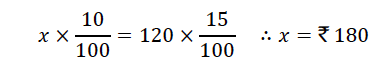Market value of 1 share = ₹ 180

(b)

 Marks No. of students fx 5 3 15 10 7 70 15 f 15f 20 9 180 25 6 150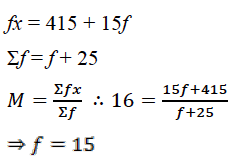(c)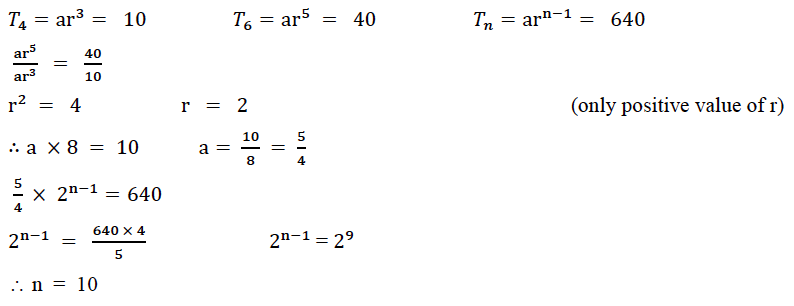### Question 6:

(a)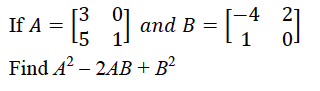(b) In the given figure AB = 9 cm, PA = 7.5 cm and PC = 5 cm. Chords AD and BC intersect at P.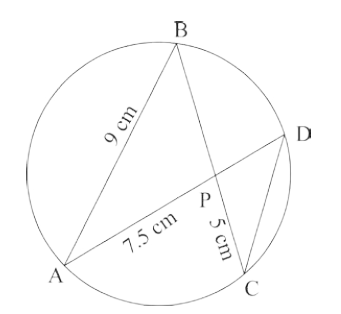(i) Prove that ΔPAB ΔPCD

(ii) Find the length of the CD.

(iii) Find area of ΔPAB: area of ΔPCD

(c) From the top of a cliff, the angle of depression of the top and bottom of a tower are observed to be 45 and 60°, respectively. If the height of the tower is 20 m.

Find:

(i) the height of the cliff

(ii) the distance between the cliff and the tower.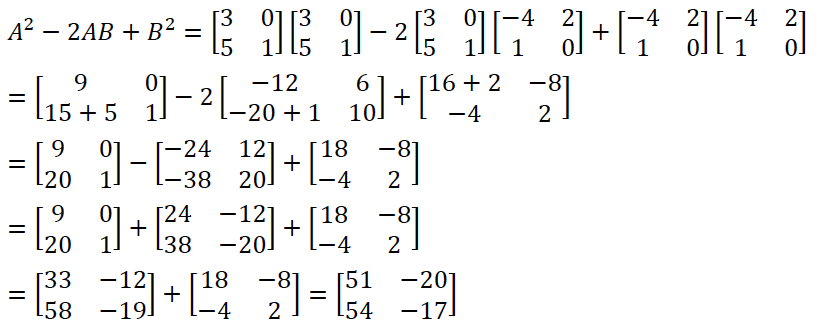(b) (i) In ΔPAB and ΔPCD

∠B = ∠D (angles in the same segment are equal)

∠APB = ∠CPD (vertically opposite angles)

∠PAB = ∠PCD (angles in the same segment are equal)

\ΔPAB ~ ΔPCD (AAA)

(ii) Since the 2 triangles are ~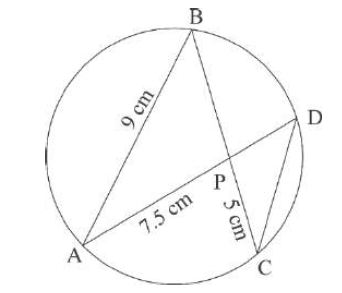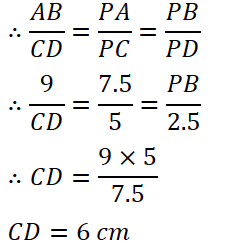(iii) ΔPAB: ΔPCD = (7.5)2 : 52

= 1.52 : 12

= 9 : 4 (CAO)

(c)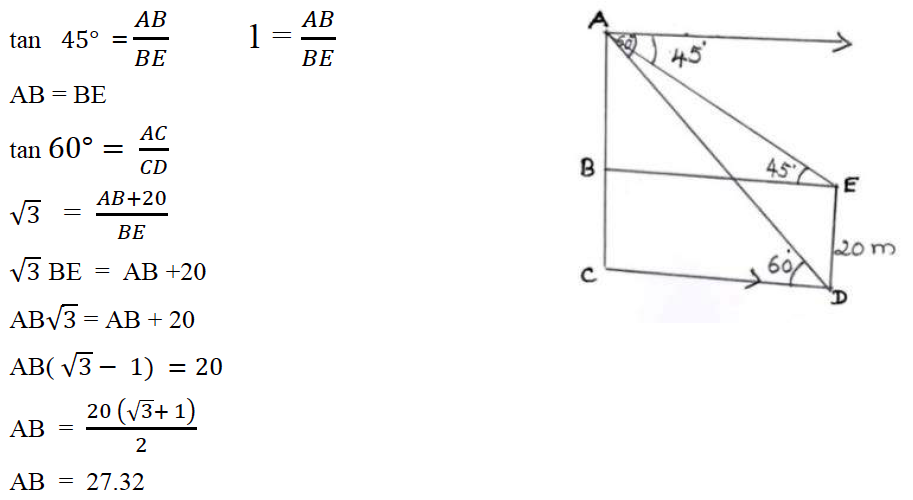(i) Height of cliff = 27.32 + 20 = 47.32 m

(ii) Distance between cliff and tower = 27.32m OR 47.3 m and 27.3 m

### Question 7:

(a) Find the value of ‘p’ if the lines, 5𝑥𝑥 − 3𝑦𝑦 + 2 = 0 and 6𝑥 − 𝑝y + 7 = 0 are perpendicular to each other. Hence find the equation of a line passing through (–2, –1) and parallel to 6𝑥−𝑝y+7=0.

(b) Using properties of proportion find 𝑥 ∶ 𝑦 , given: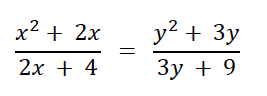(c) In the given figure TP and TQ are two tangents to the circle with centre O, touching at A and C, respectively. If ∠BCQ = 55o and ∠BAP = 60o, find:

(i) ∠OBA and ∠OBC

(ii) ∠AOC

(iii) ∠ATC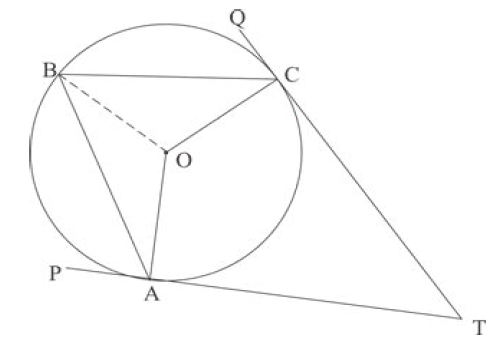5𝑥 − 3𝑦 + 2 = 0 ∴ slope = 5/3

6𝑥 − 𝑝y + 7 = 0 ∴ slope = 6/𝑝

∵ they are right angles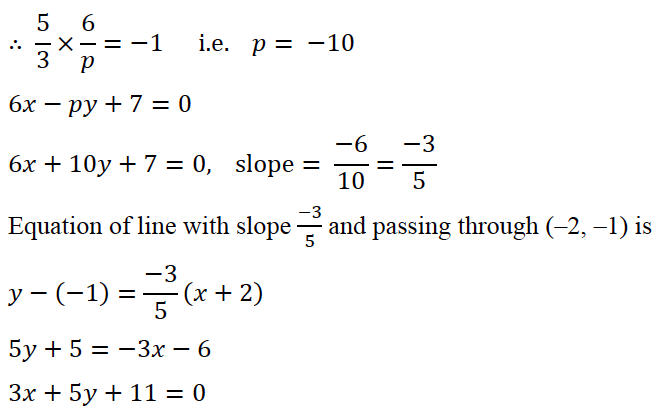(b)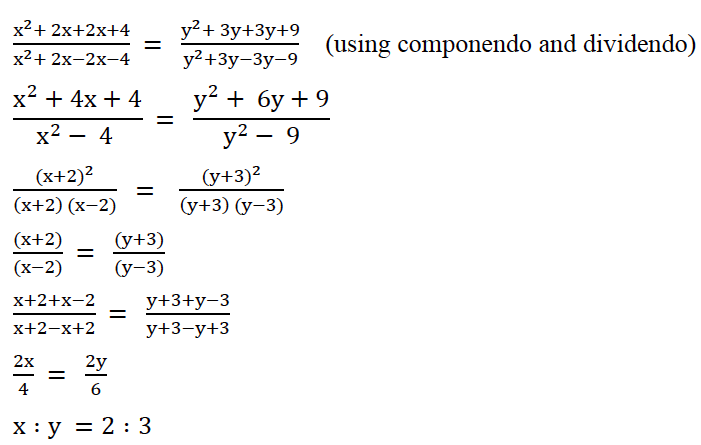(c) (i) ∠BCQ = 55o

∠OCB = 90o – 55o = 35o (radius & tangent makes ∠ = 90o)

∠OBC = ∠OCB = 35o ( OB = OC)

∠OBA = ∠OAB = 90o – 60o = 30o ( OA = OB & ∠OAP = 90o)

∠OBA = 30o

(ii) ∠ABC = ∠OBA + ∠OCB = 30o + 35o = 65o

∠AOC = 2∠ABC = 2 x 65o = 130o (Angle at the centre is double angle at the

∠AOC = 130o remaining circumference)

(iii) ∠ATC = 360o – (90 + 90 + ∠AOC) ( angles of quadrilateral adds up to 180o)

∠ATC = 360o – (180o + 130o)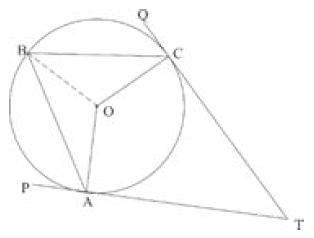= 360o – 310o

= 50o

### Question 8:

(a) What must be added to the polynomial 2𝑥𝑥3 − 3𝑥𝑥2 − 8𝑥𝑥, so that it leaves a remainder 10 when divided by 2x + 1?

(b) Mr. Sonu has a recurring deposit account and deposits ₹ 750 per month for 2 years. If he gets ₹ 19125 at the time of maturity, find the rate of interest.

(c) Use graph paper for this question. Take 1 cm = 1 unit on both x and y axes.

(i) Plot the following points on your graph sheets:

A (–4, 0), B (–3, 2), C (0, 4), D (4, 1) and E (7, 3)

(ii) Reflect the points B, C, D and E on the x-axis and name them as B’, C’, D’ and E’ respectively.

(iii) Join the points A, B, C, D, E, E’, D’, C’, B’ and A in order.

(iv) Name the closed figure formed.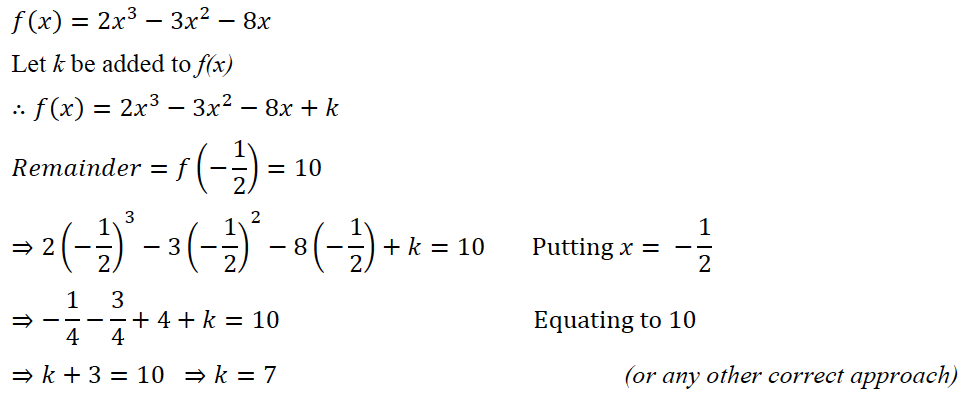(b)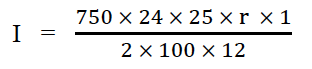= 375 r/2

Amount deposit = 750 × 24 = Rs 18000

Maturity value = Rs 19125

Interest = Rs 19125 – 18000 = Rs 1125

1125 = 375 r/2

𝐫 = 6 %

(c) (i) Plotted the points A,B,C,D and E correctly.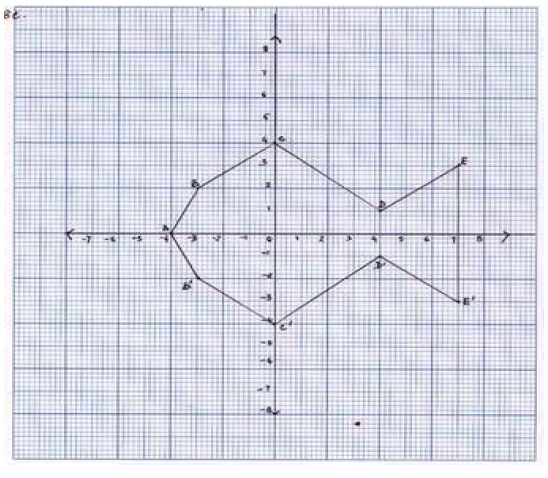(ii) Reflected the points B, C, D and E on the x-axis and named them as B’, C’, D’ and E’

correctly.

(iii) Joined the points A, B, C, D, E, E’, D’, C’, B’ and A in order and completed the figure.

(iv) Nine-sided polygon or nonagon, polygon fish or kite.

### Question 9:

(a) 40 students enter for a game of shot-put competition. The distance thrown (in metres) is recorded below:

 Distance in m 12-13 13-14 14-15 15-16 16-17 17-18 18-19 Number of Students 3 9 12 9 4 2 1

Use a graph paper to draw an ogive for the above distribution.

Use a scale of 2 cm = 1 m on one axis and 2 cm = 5 students on the other axis.

(i) the median

(ii) Upper Quartile

(iii) Number of students who cover a distance which is above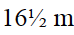.

(b) If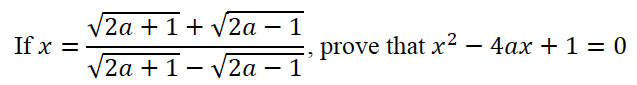Distance in m Number of students Cumulative frequency (c.f.) 12-13 3 3 13-14 9 12 14-15 12 24 15-16 9 33 16-17 4 37 17-18 2 39 18-19 1 40

(i) Median = 14.7 m ± 0.2

(ii) Upper Quartile = 15.65 m ± 0.2

(iii) 40 – 35 = 5 students cover a distance above.

Ogive: Scale 2 cm = 1m along x-axis and 2 cm = 5 students along y-axis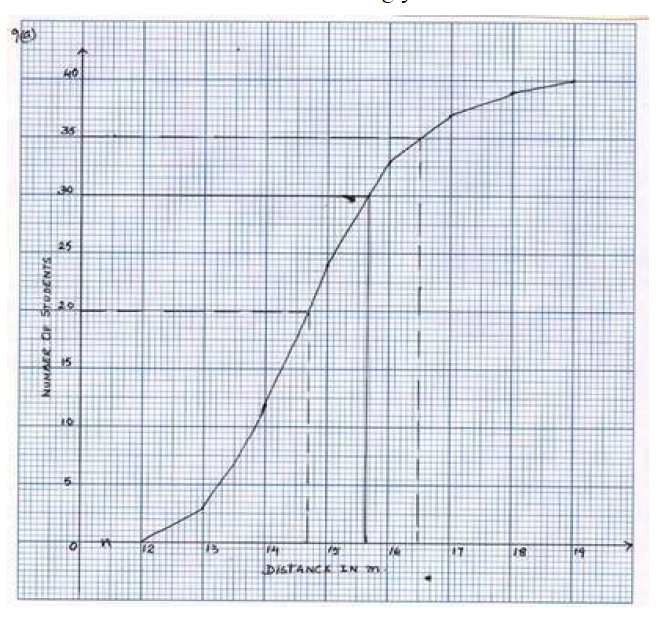(b) Using Componendo / Dividendo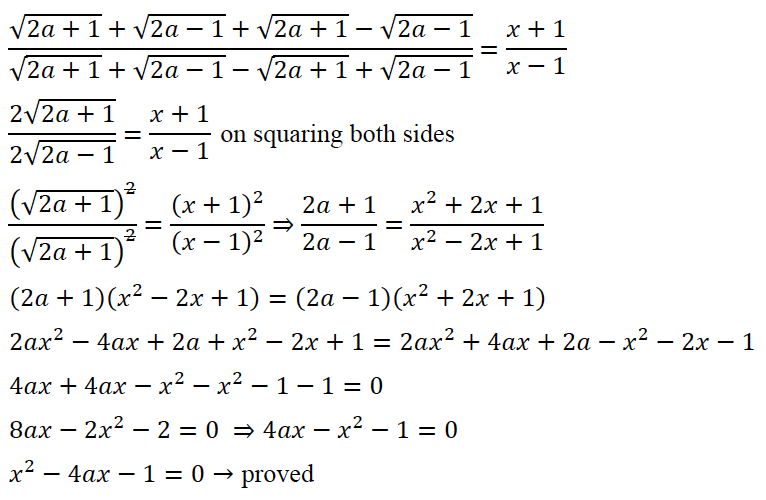### Question 10:

(a) If the 6th term of an A.P. is equal to four times its first term and the sum of first six terms is 75, find the first term and the common difference.

(b) The difference of two natural numbers is 7 and their product is 450. Find the numbers.

(c) Use ruler and compass for this question. Construct a circle of radius 4.5 cm. Draw a chord. AB = 6 cm.

(i) Find the locus of points equidistant from A and B. Mark the point where it meets the circle as D.

(ii) Join AD and find the locus of points which are equidistant from AD and AB. Mark the point where it meets the circle as C.

(iii) Join BC and CD. Measure and write down the length of the side CD of the quadrilateral ABCD.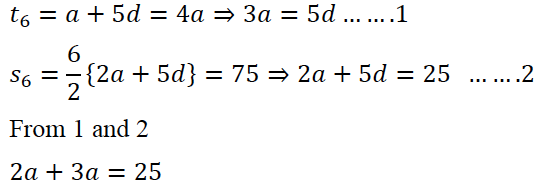⇒ 𝑎 = 5

𝑑= 3

(b) Let the numbers be x and (𝑥 + 7)

𝑥(𝑥 + 7) = 450

𝑥2 + 7𝑥 − 450 = 0

(𝑥 + 25) (𝑥 − 18) = 0

𝑥 = −25 or 18

The numbers are 18 and 25. (Any other correct method)

(c) Circle and chord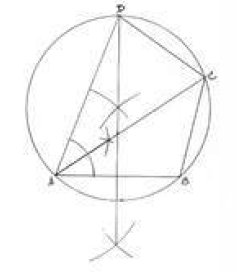(i) Bisector of AB and point D (any side)

(ii) Bisector of ∠BAD and point C (any side).

(iii) Length of CD = 5 cm (±.3)

OR CD = 1.4 (±0.3). If C and D are points below AB.

### Question 11:

(a) A model of a high rise building is made to a scale of 1 : 50.

(i) If the height of the model is 0.8 m, find the height of the actual building.

(ii) If the floor area of a flat in the building is 20 m2, find the floor area of that in the model.

(b) From a solid wooden cylinder of height 28 cm and diameter 6 cm, two conical cavities are hollowed out. The diameters of the cones are also of 6 cm and height 10.5 cm.

Taking π = 22/7 find the volume of the remaining solid.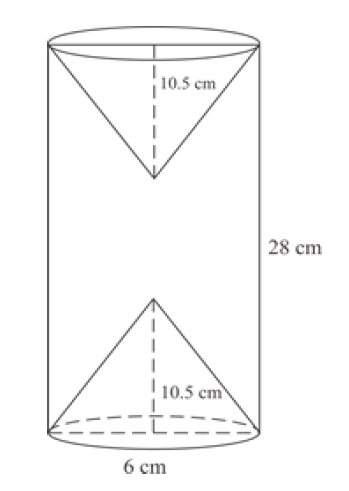(c) Prove the identity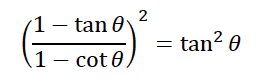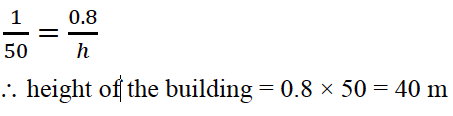(ii)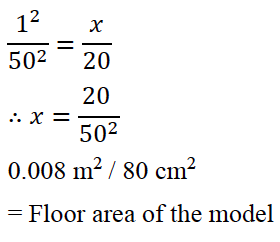(b) Volume of Remaining Solid = Volume of Cylinder – 2 × Volume of Cone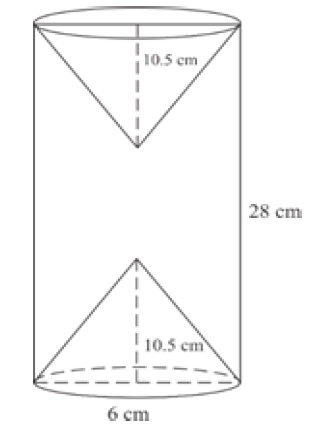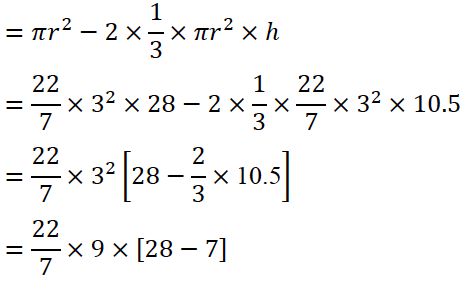= 22/7 × 9 × 21

= 66 × 9

= 594 cm3

(c)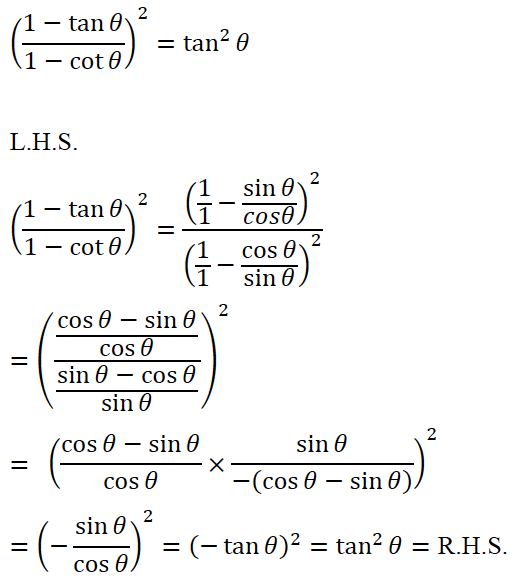Alternative Method: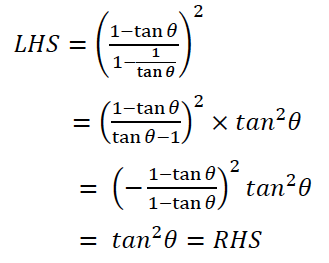NOTE: For questions having more than one correct answer/solution, alternative correct answers / solutions, apart from those given in the marking scheme, have also been accepted.

We hope students must have found this information on “ICSE Class 10 Maths Question Papers Solutions 2020” helpful for their studies. To get the year-wise ICSE Class 10 Previous Years Question papers along with solutions for other subjects, click here. Keep learning and download the BYJU’S App to access interactive study videos.